# Tamil Nadu Board Question Papers for Class 12th Maths 2018 With Solutions In PDF

Tamil Nadu Class 12 Maths 2018 question paper with solutions are provided here in a downloadable pdf format and also in the text, so that the students can get to know their weaker areas and prepare for the board exams. Along with the solutions, Maths question paper 2018 Class 12 is also added for reference. Students are able to access all the Tamil Nadu board previous year Maths question papers here.

The solutions provided by BYJU’S are prepared by experts and can be relied upon for effective guidance. Solving 2018 Maths question papers and also the previous year papers for Class 12 will make the student more confident. These solutions can be accessed with a lot of ease on the website and is an ideal way to learn and revise for the exams. The solutions are prepared in a step by step format.

In this article here, we have provided the PDF link to access the Tamil Nadu Board Class 12 Maths Previous Year Question Paper 2018 with Solutions or for just the question paper as required.

TN Board Class 12th Maths Question Paper With Solutions 2018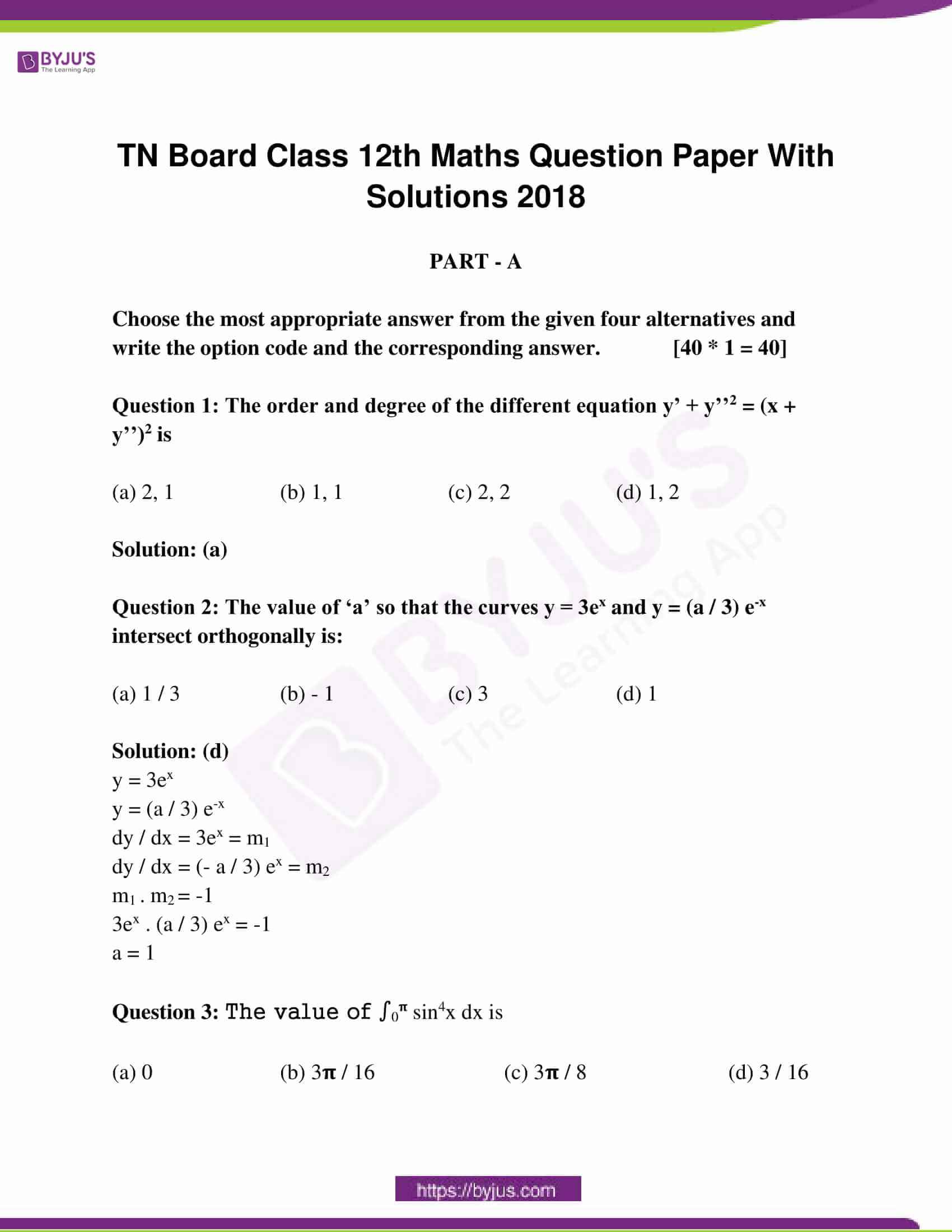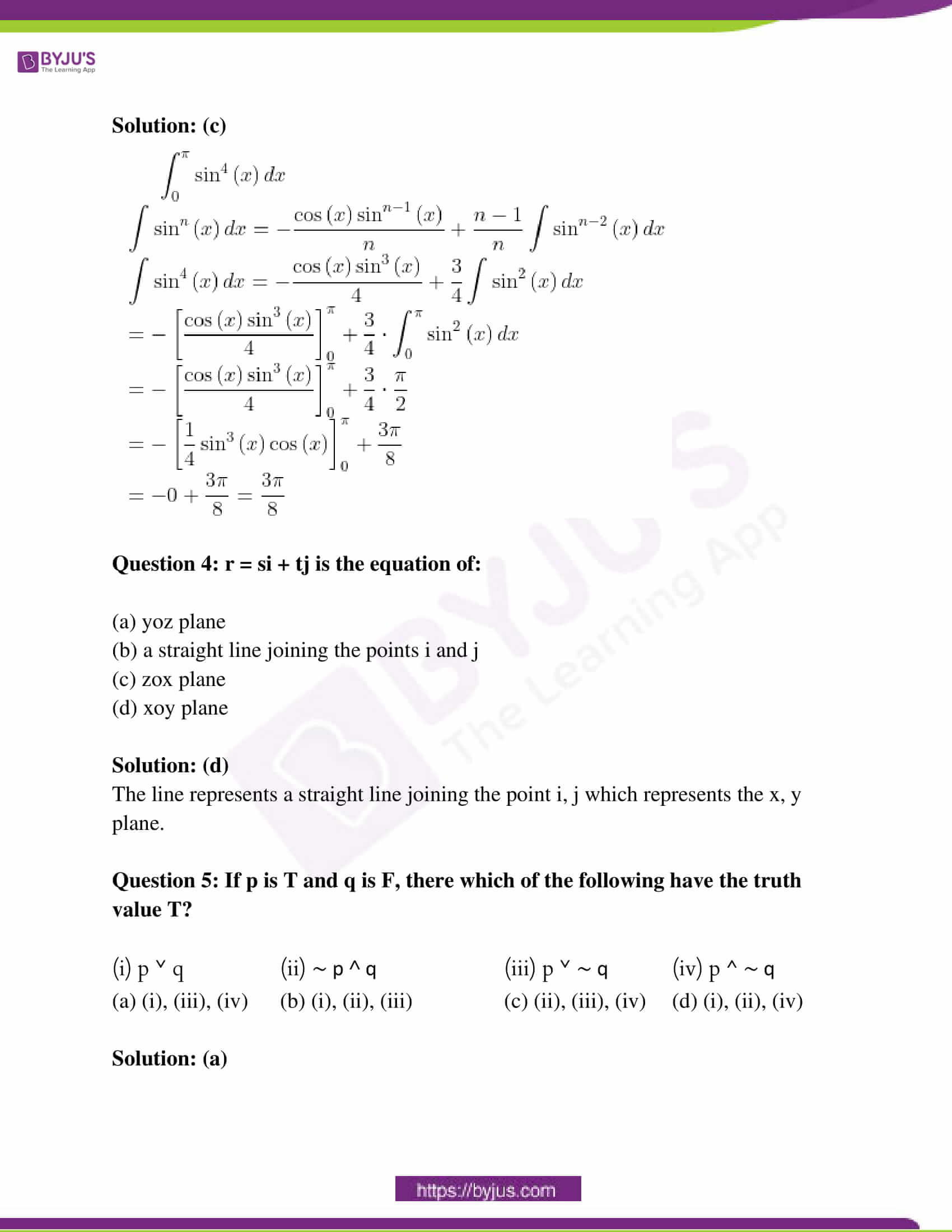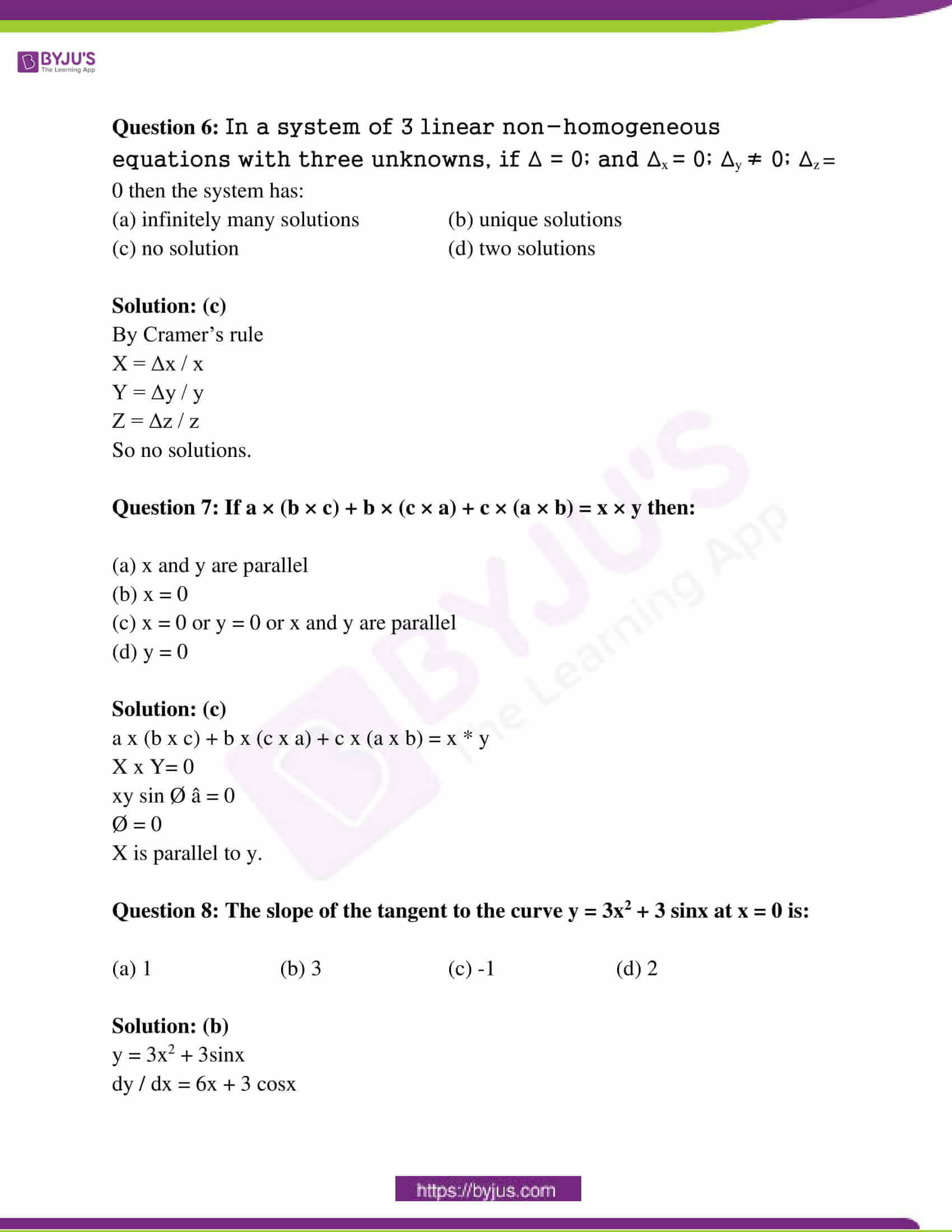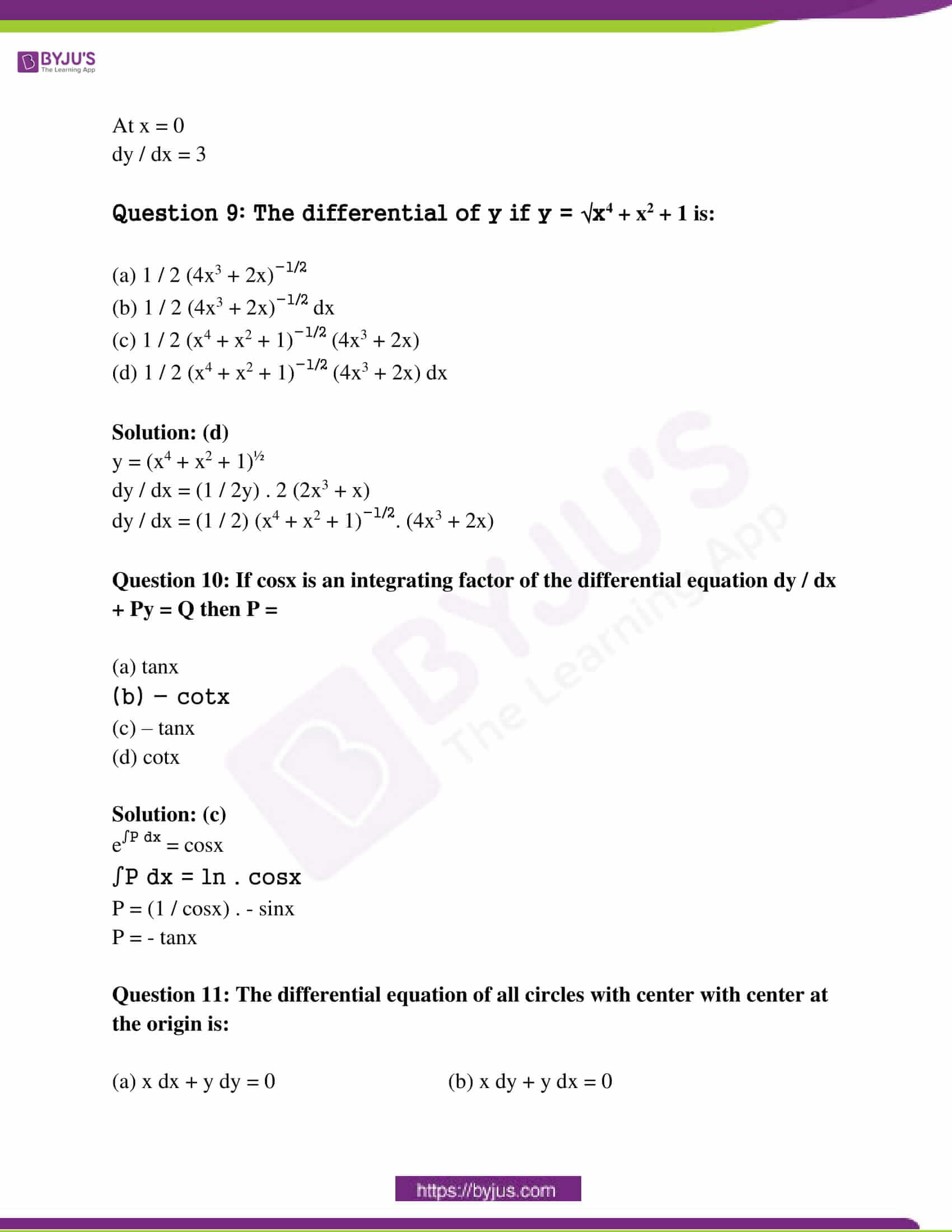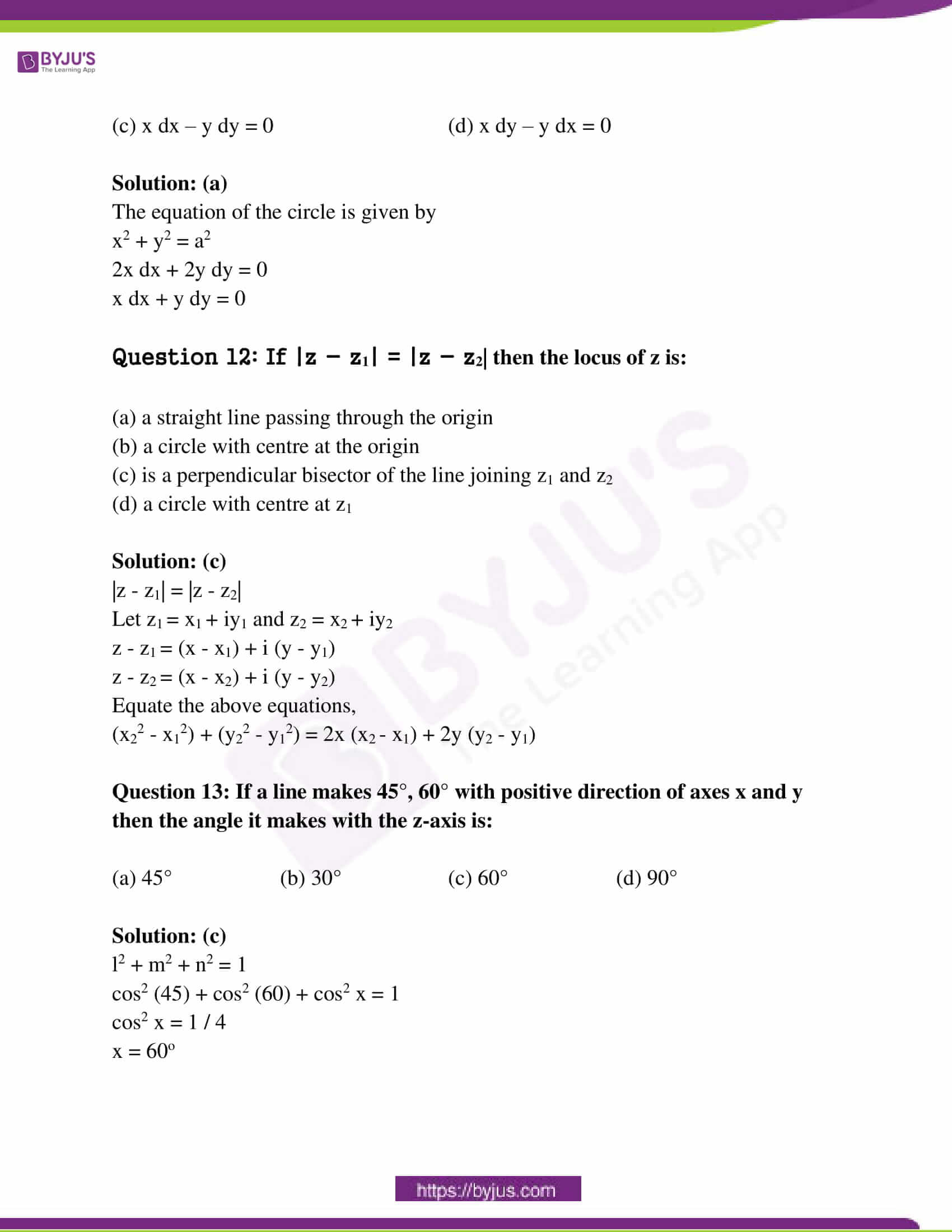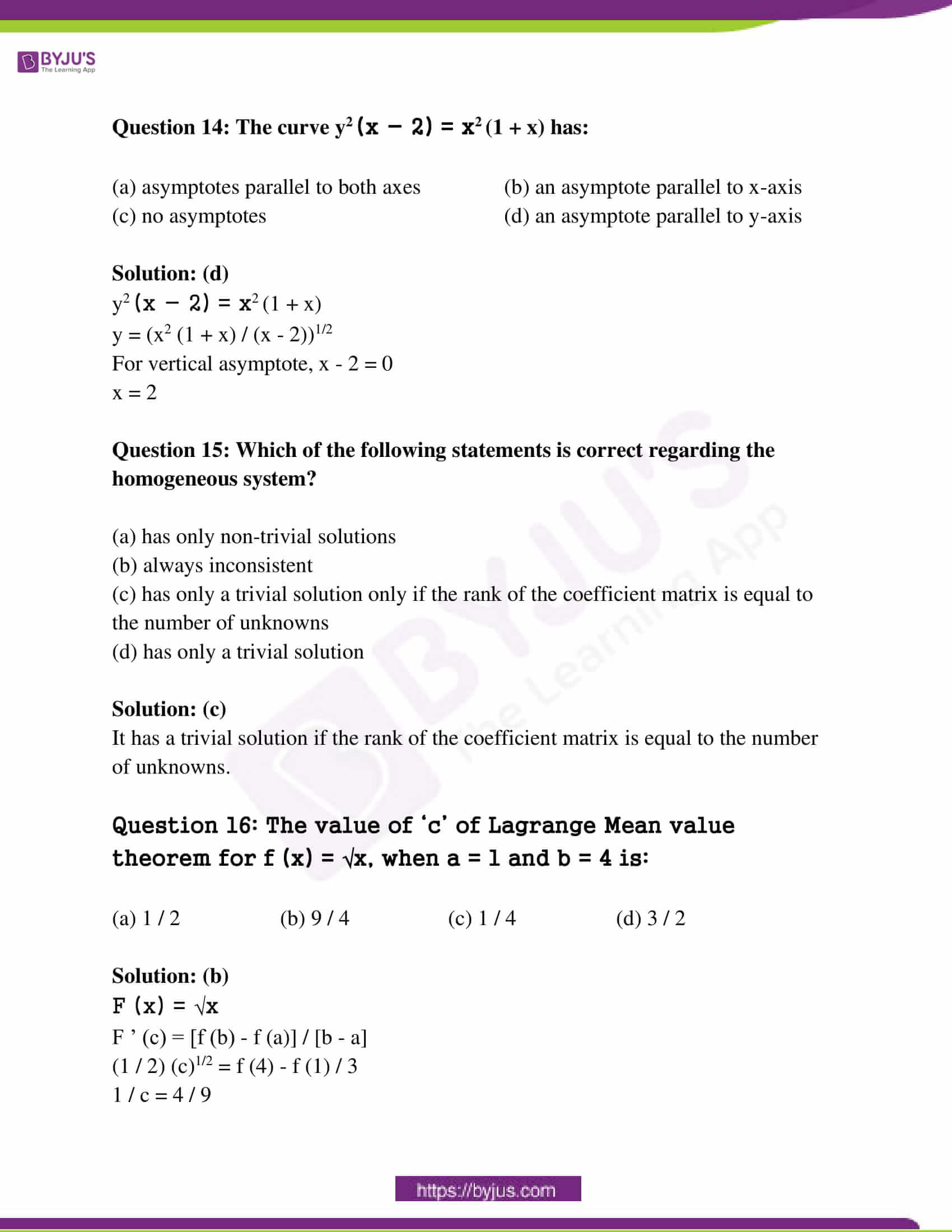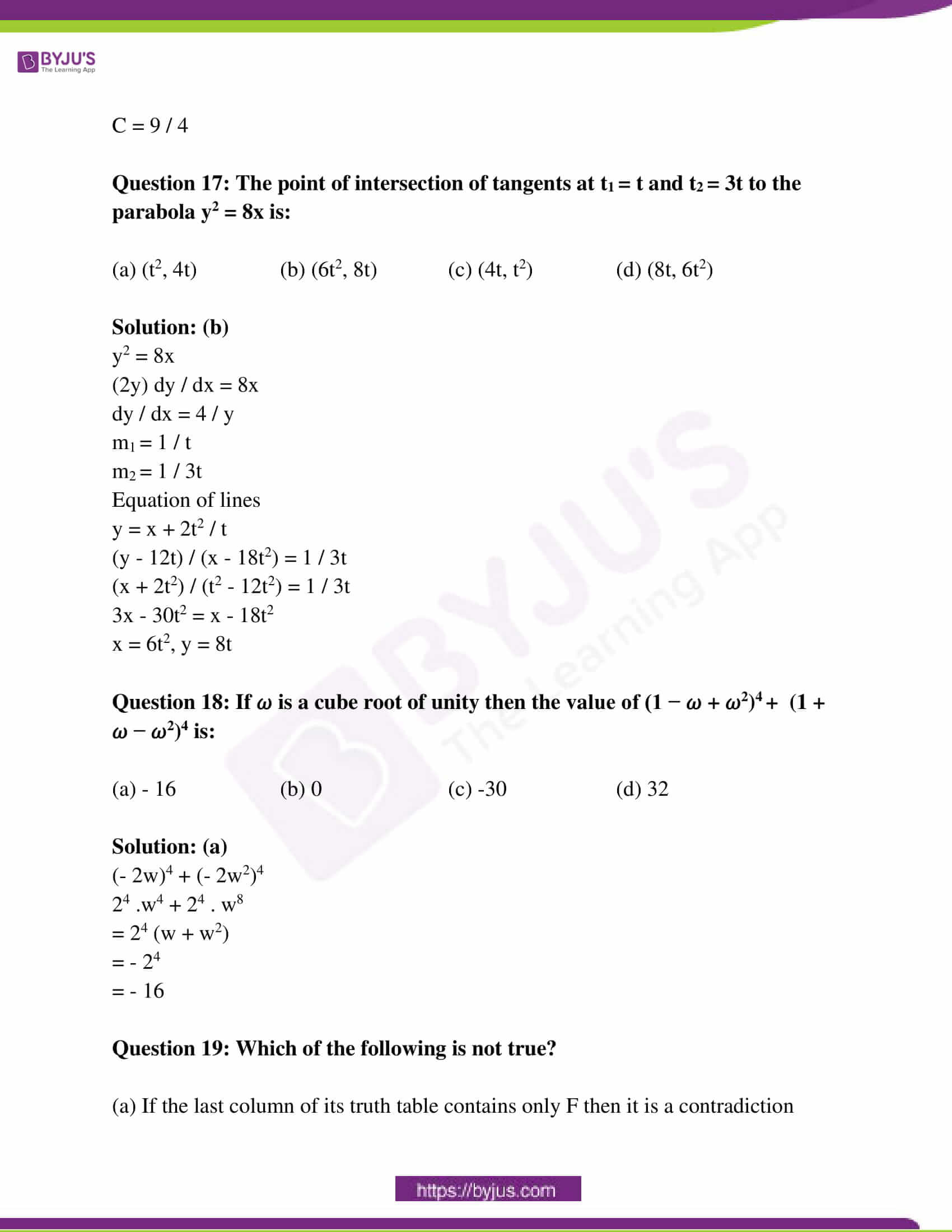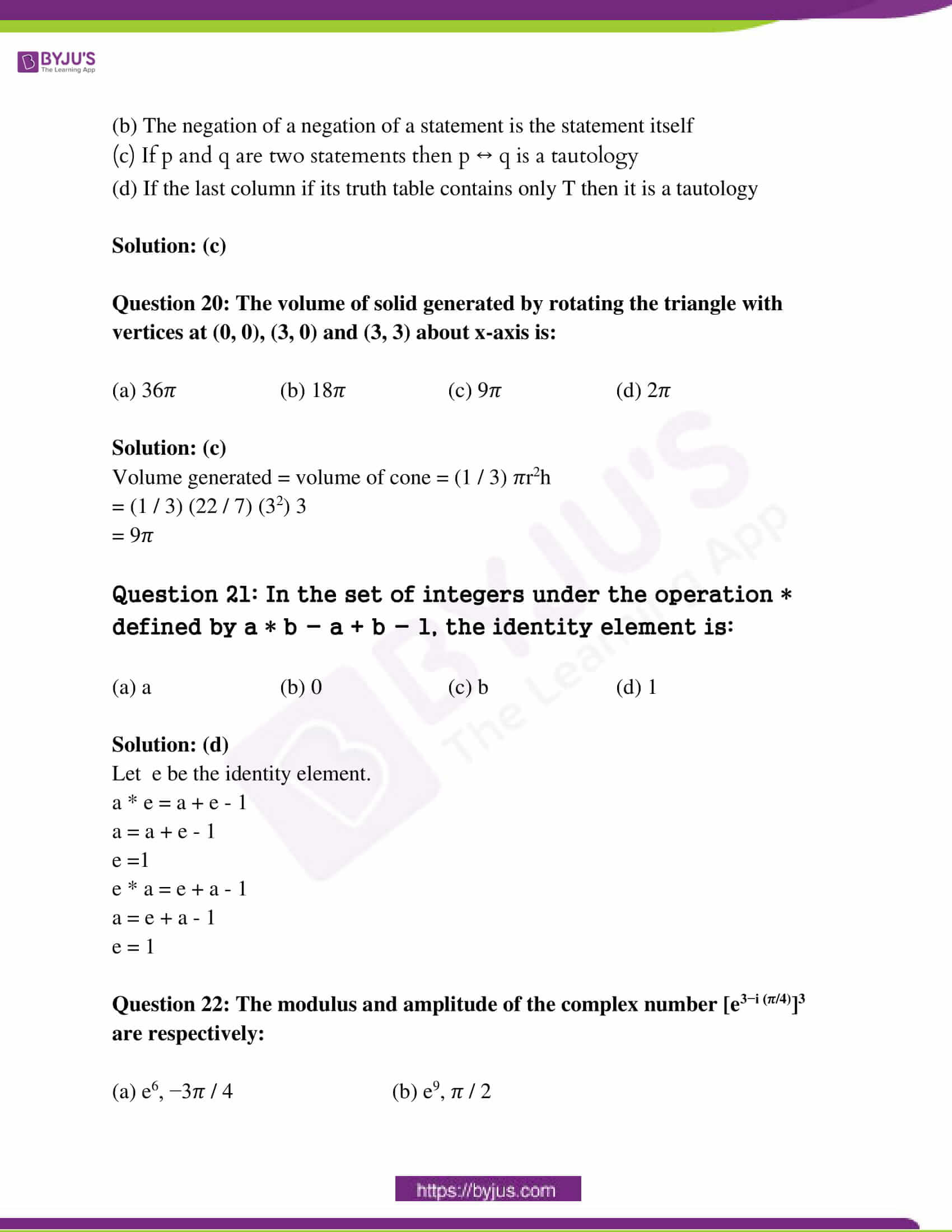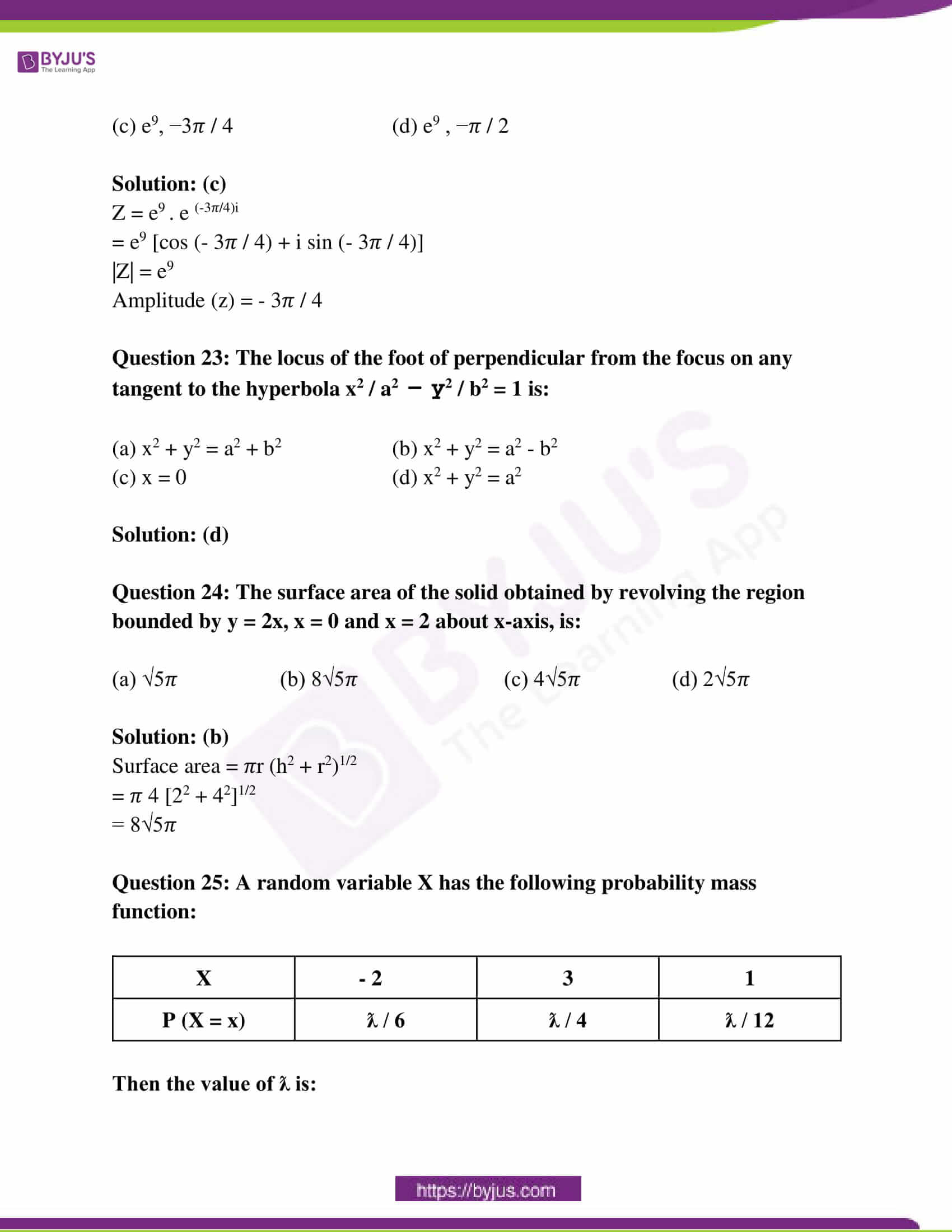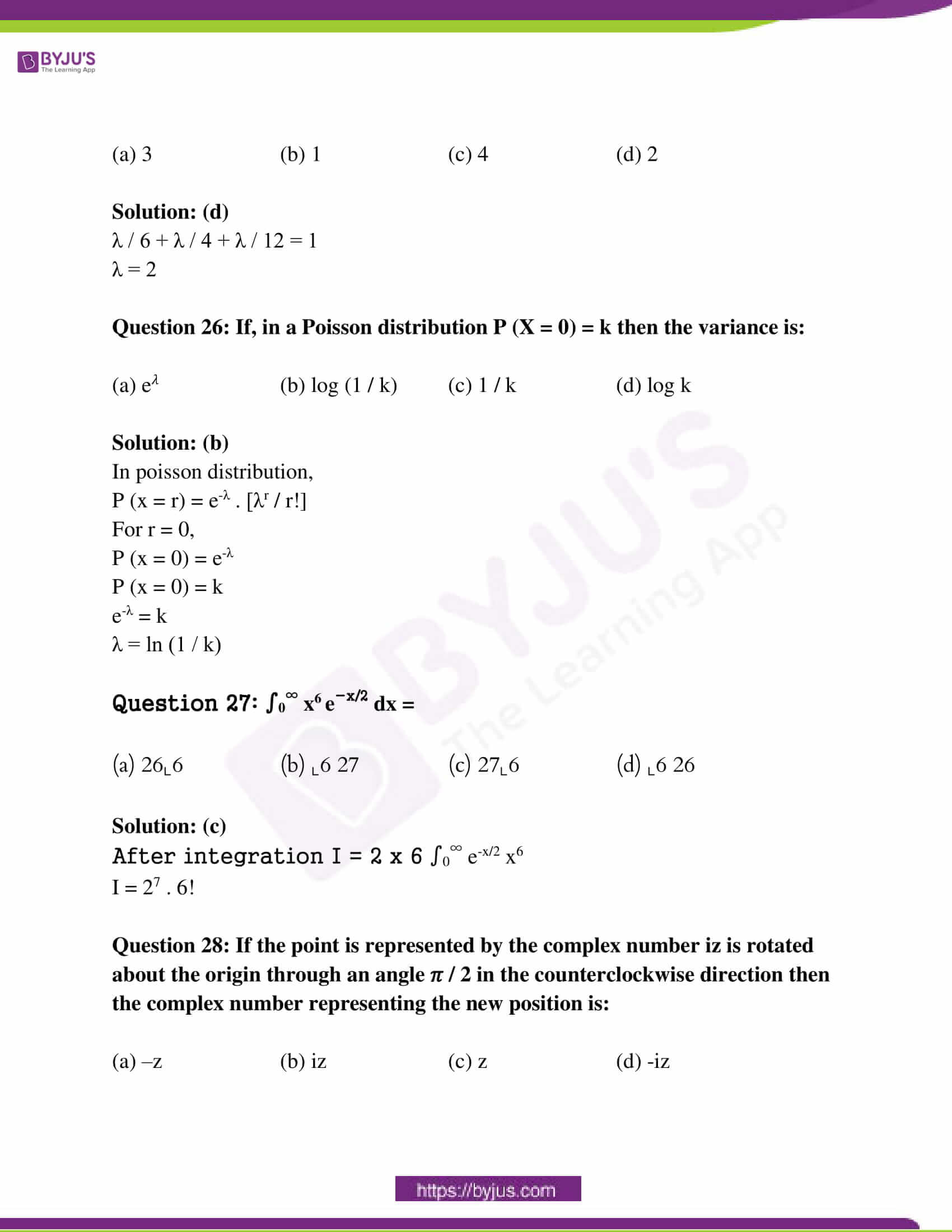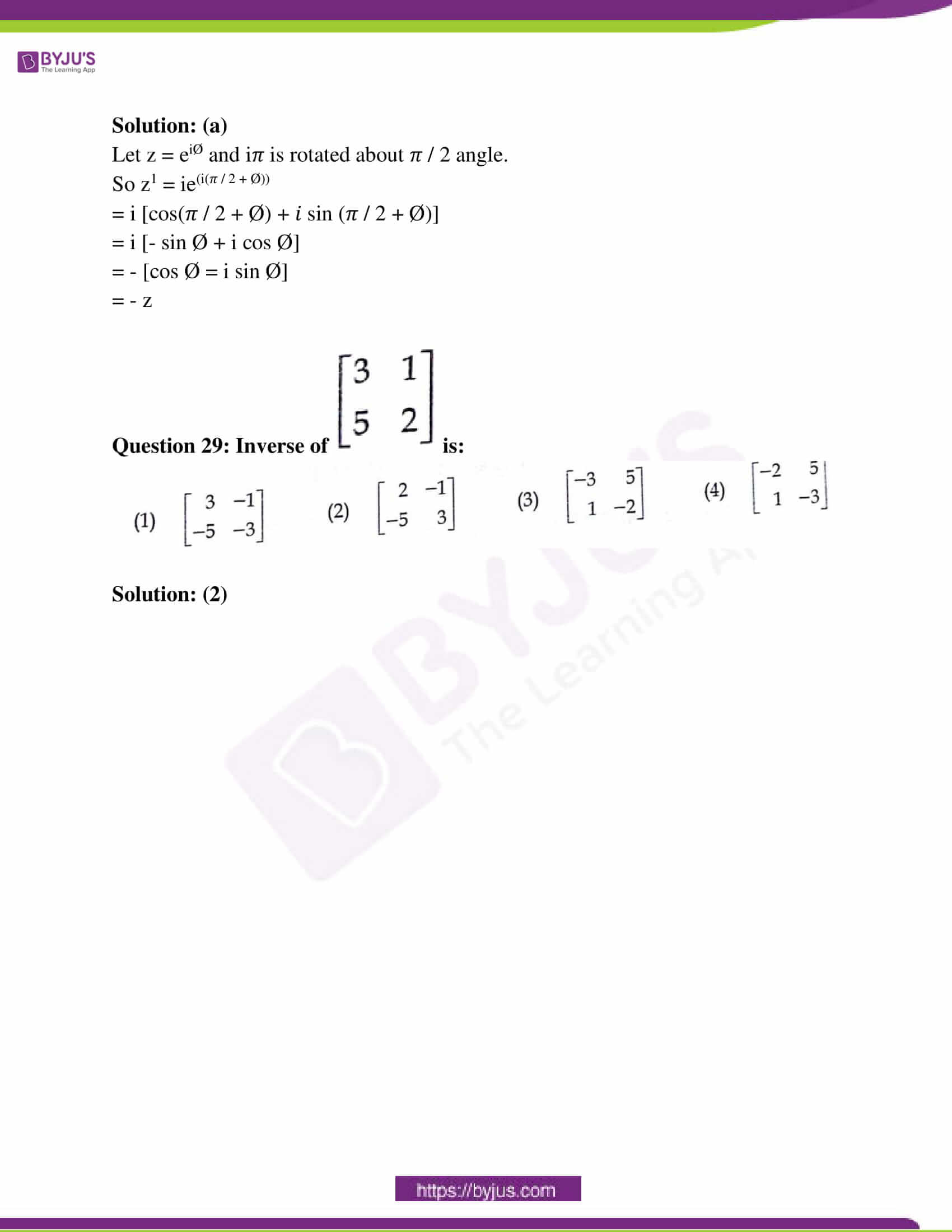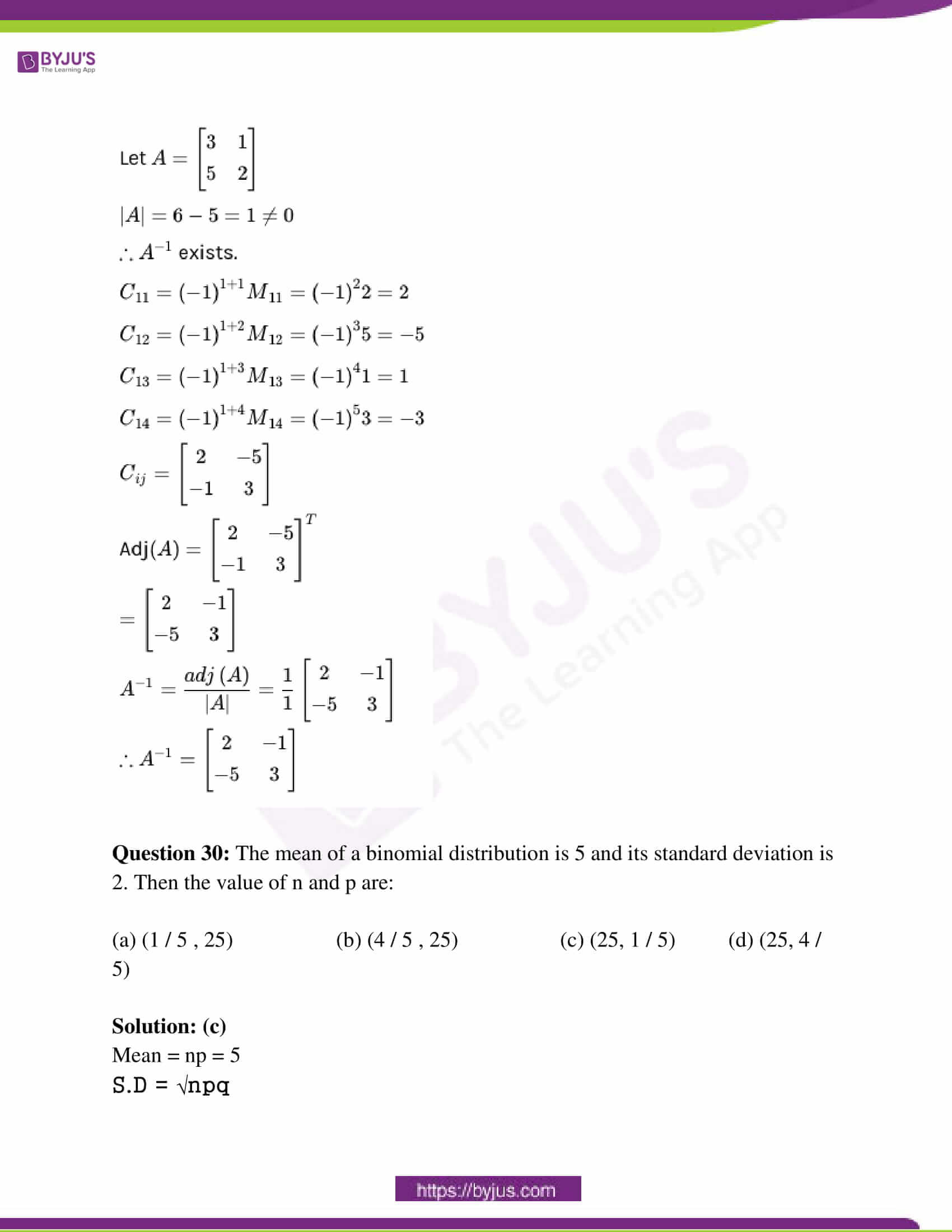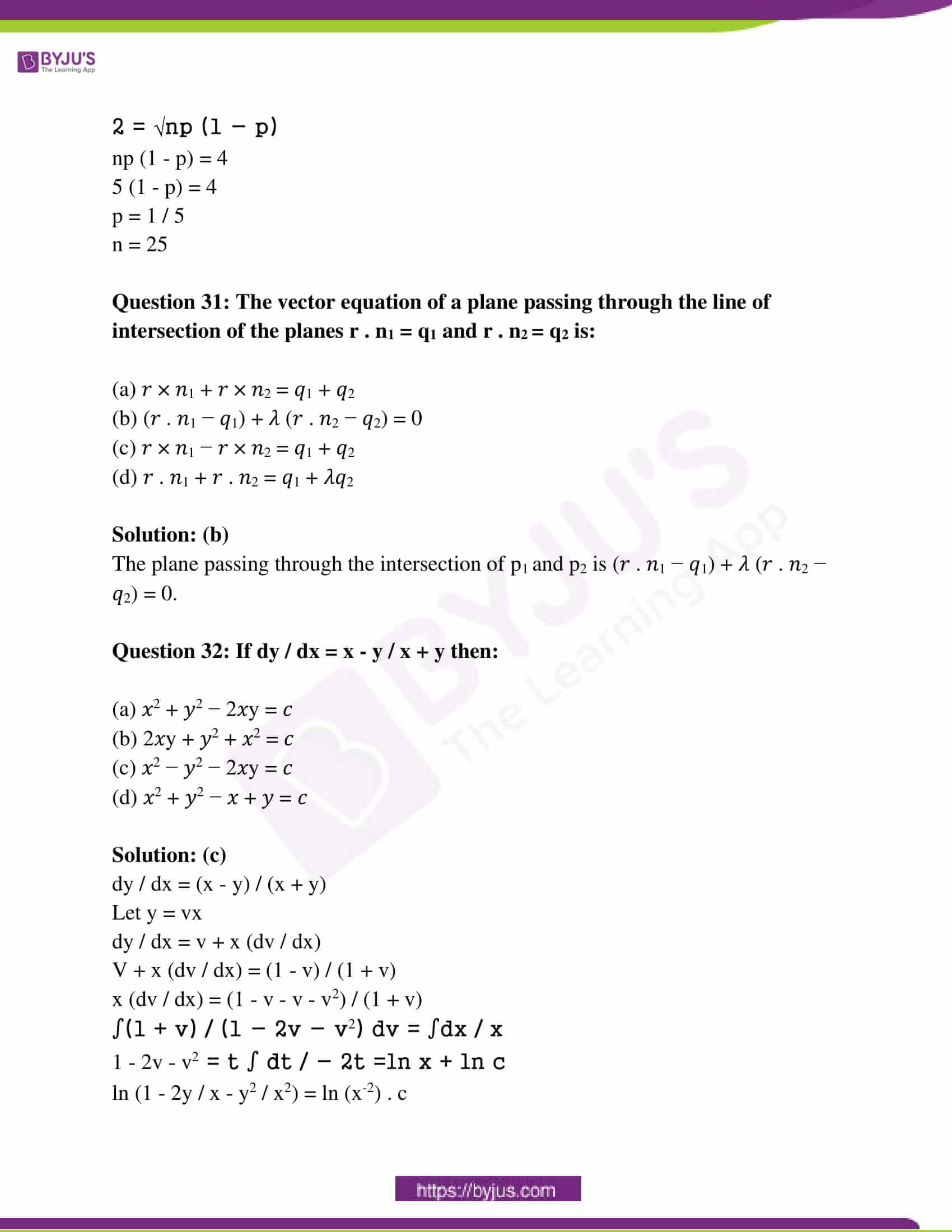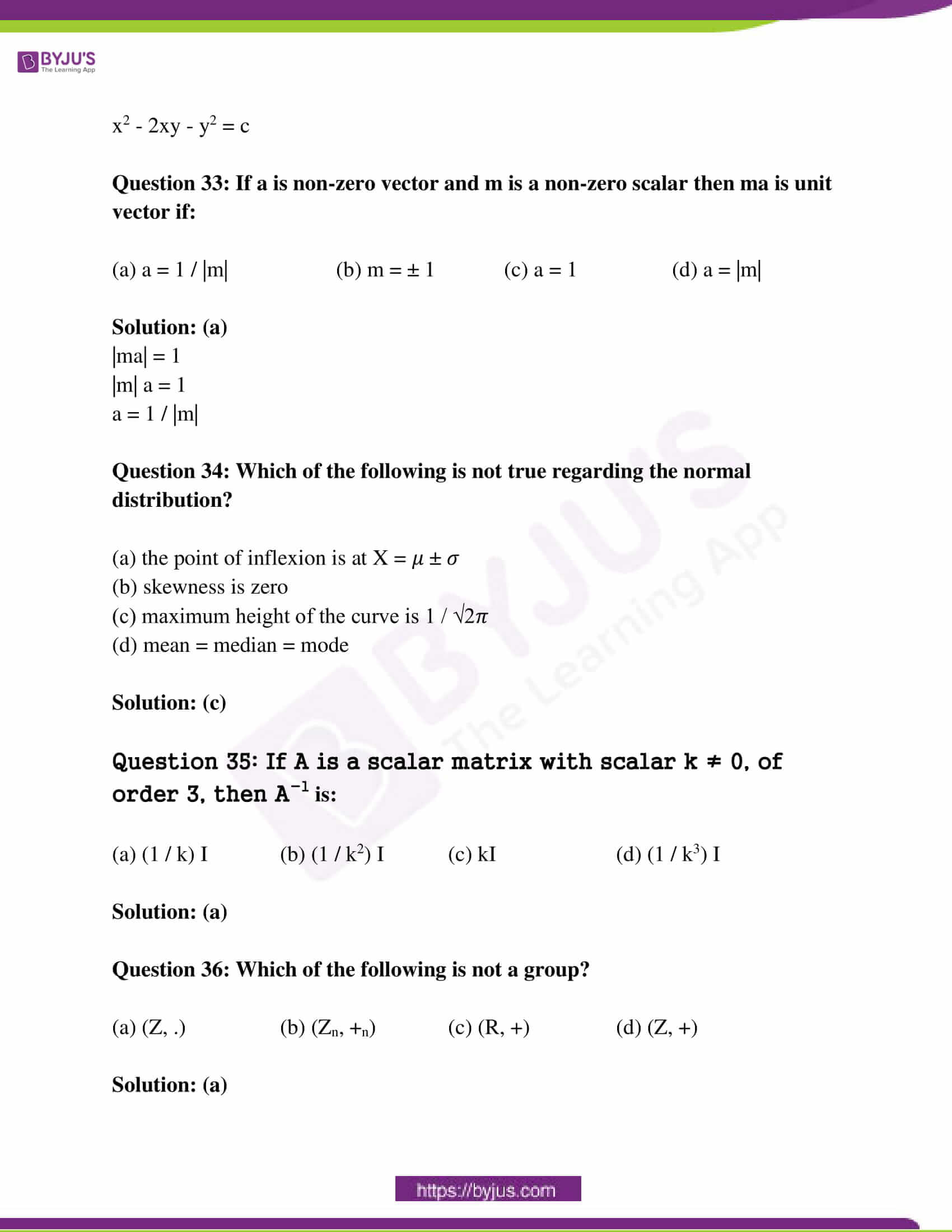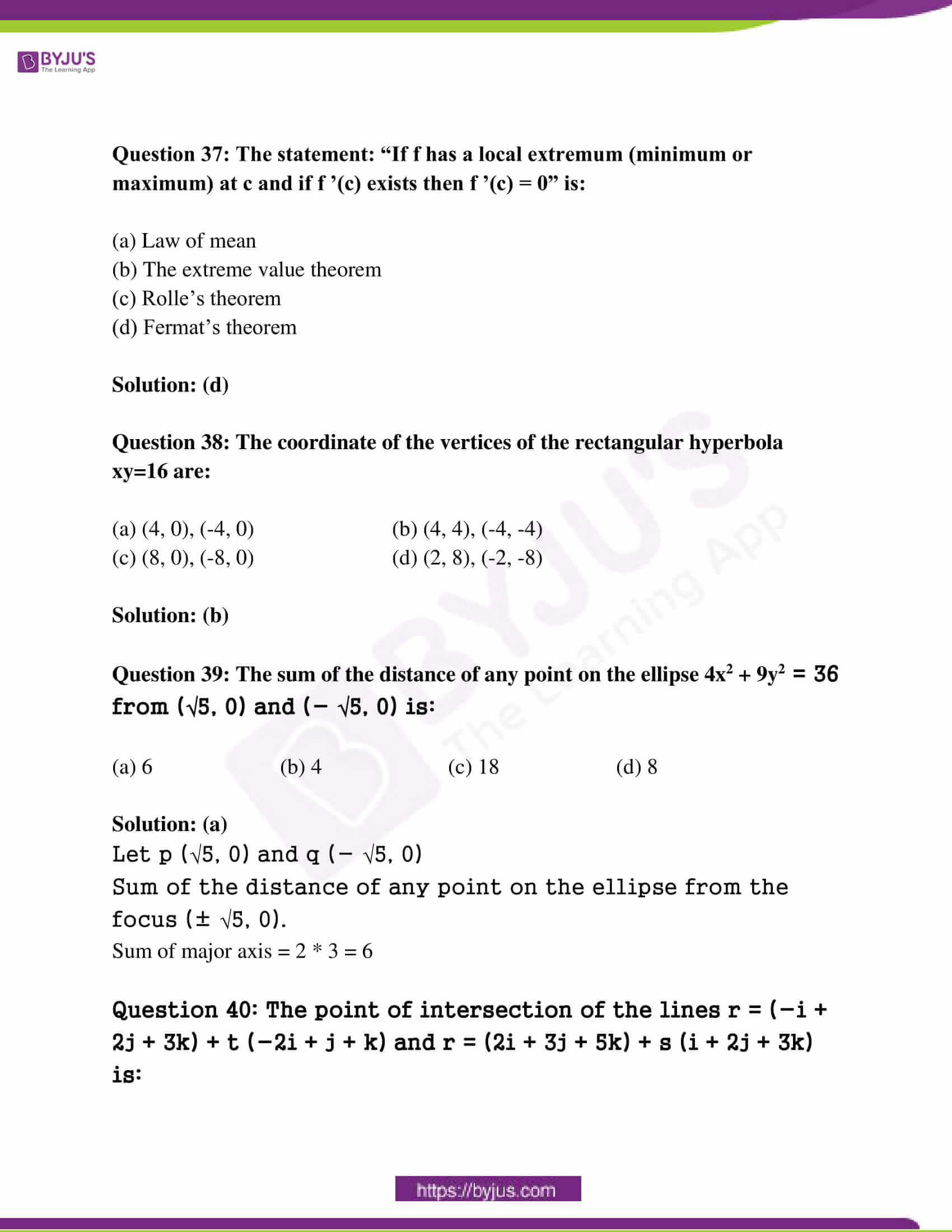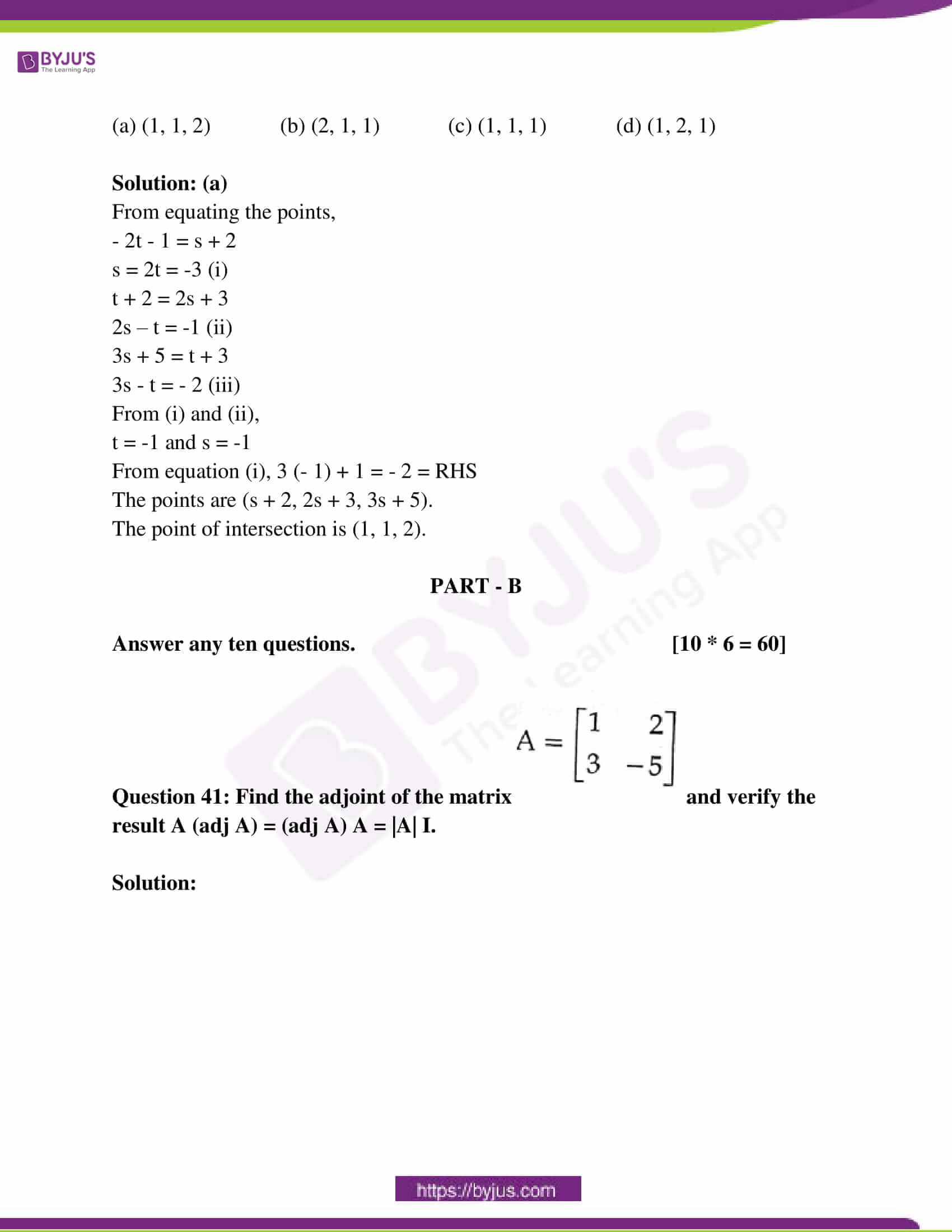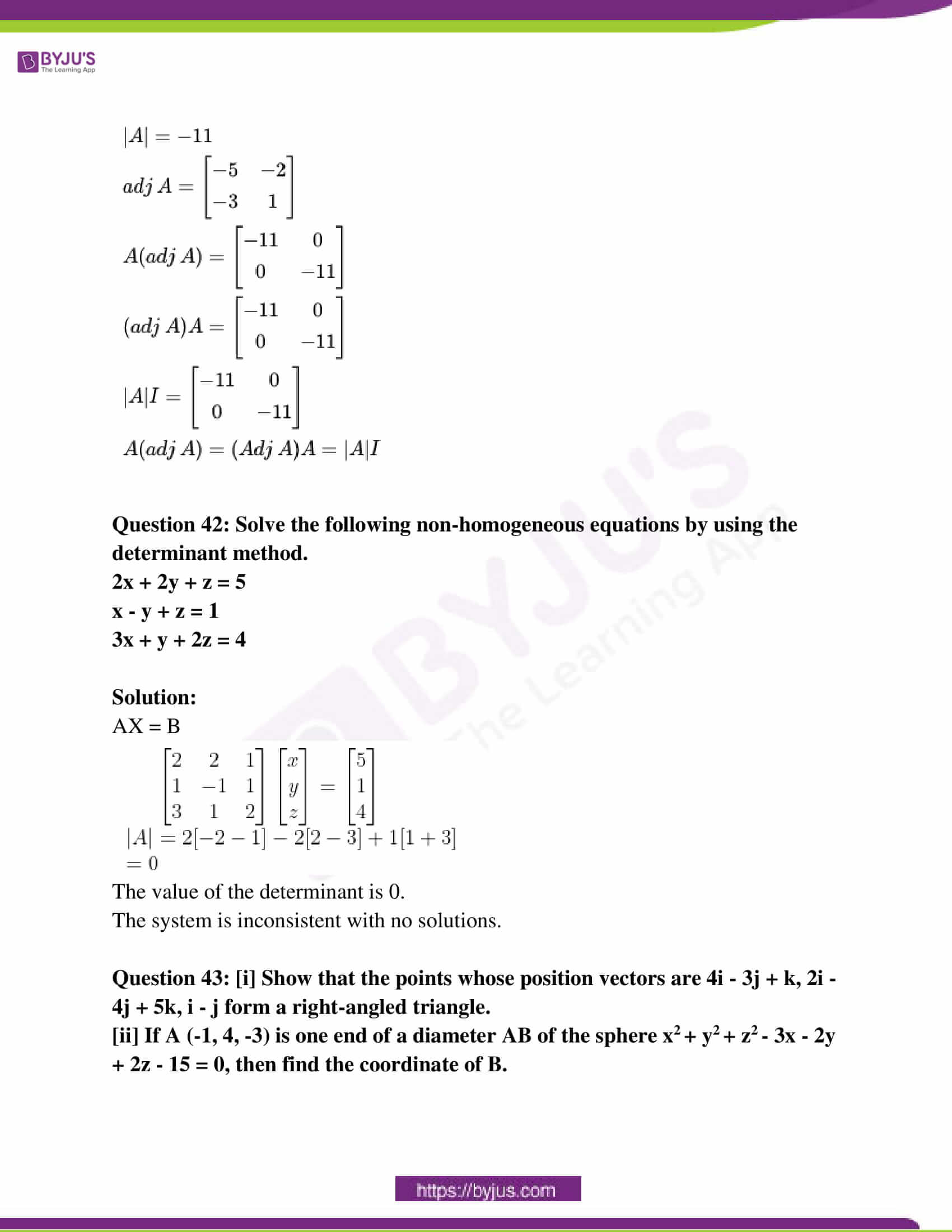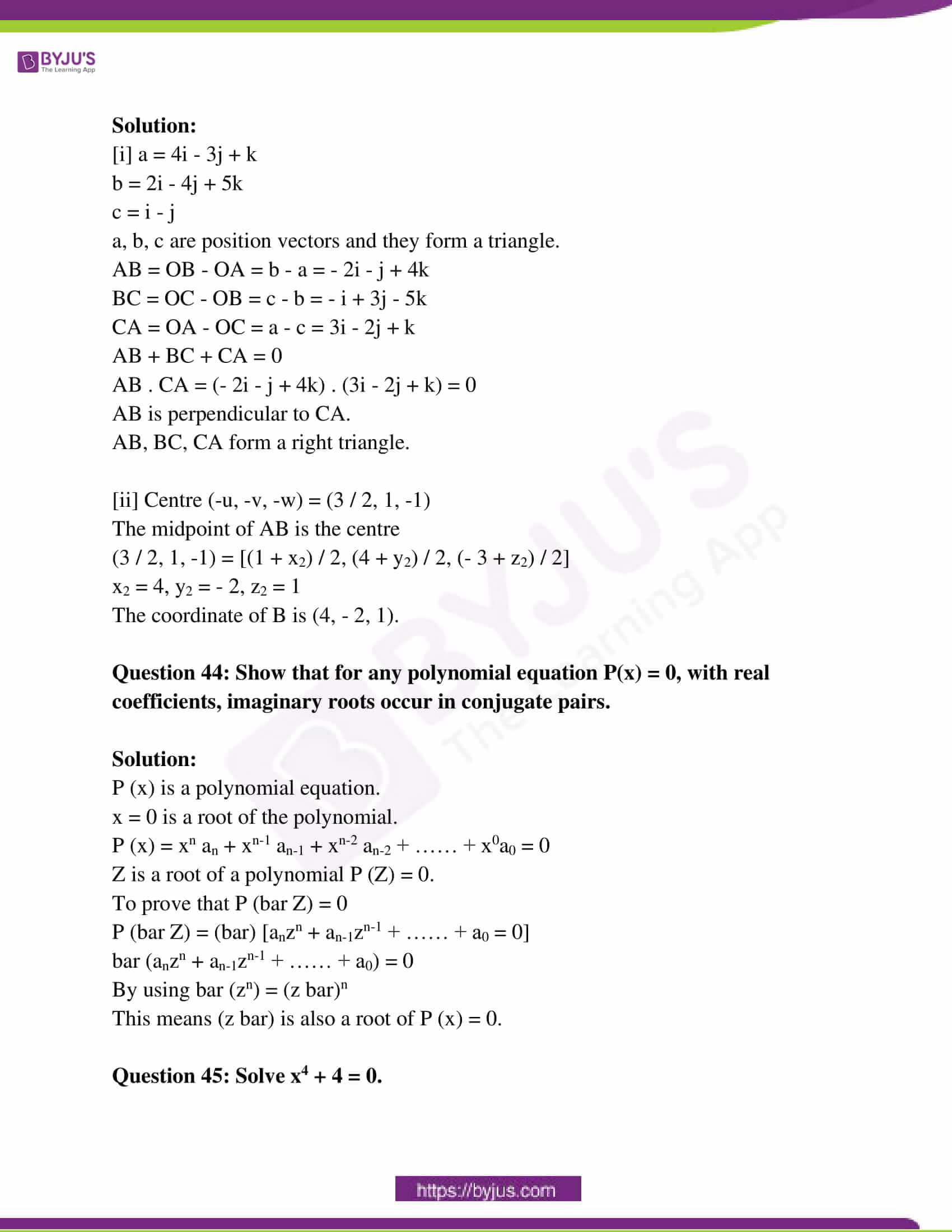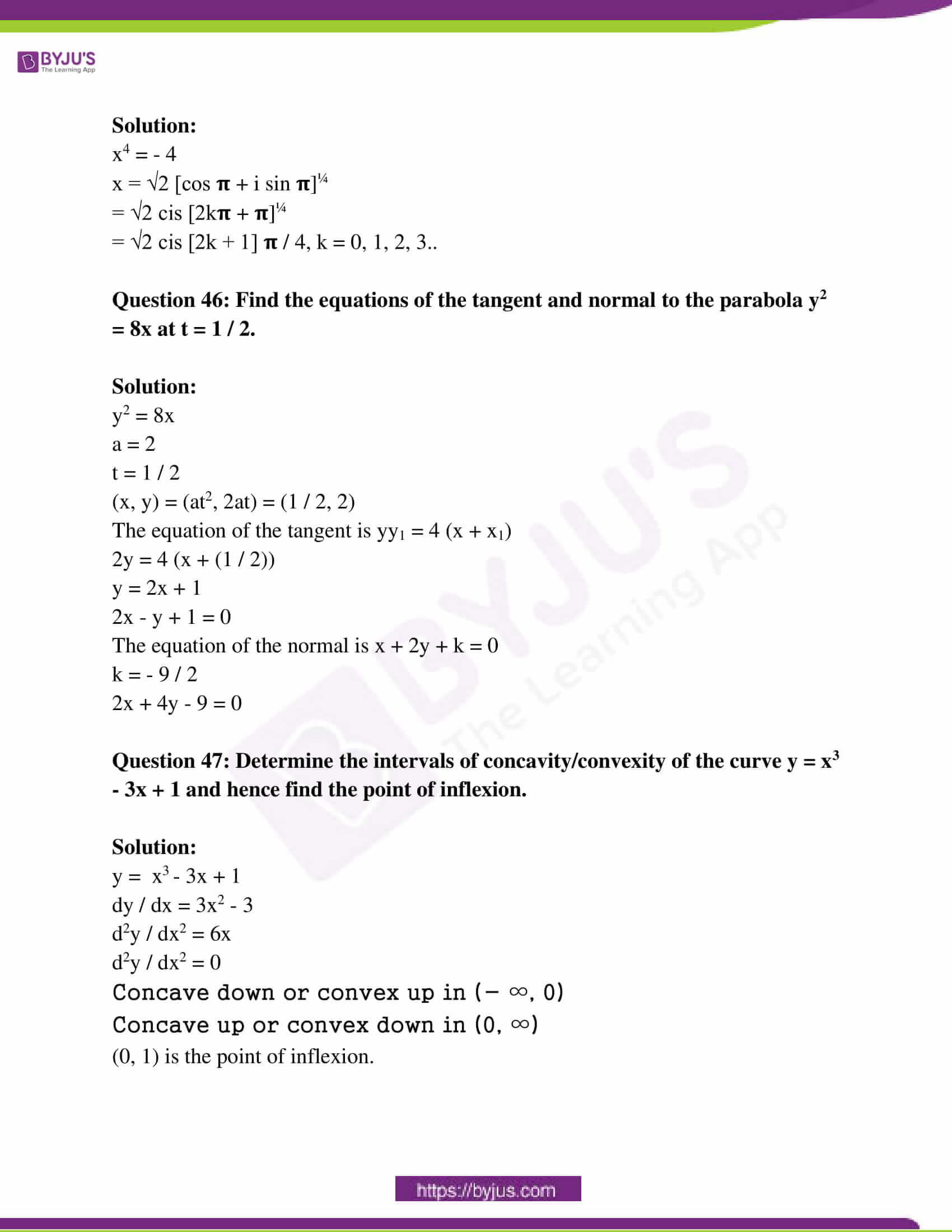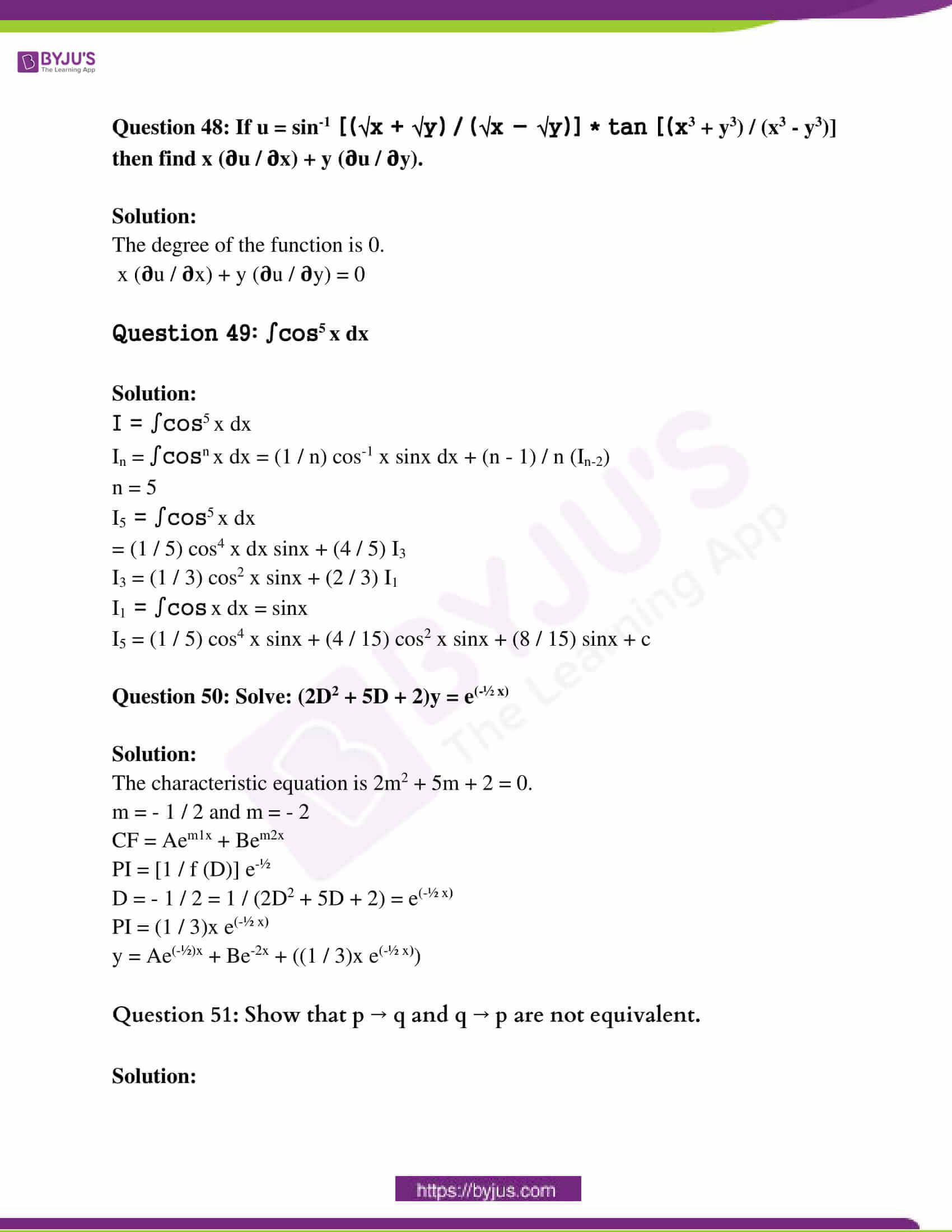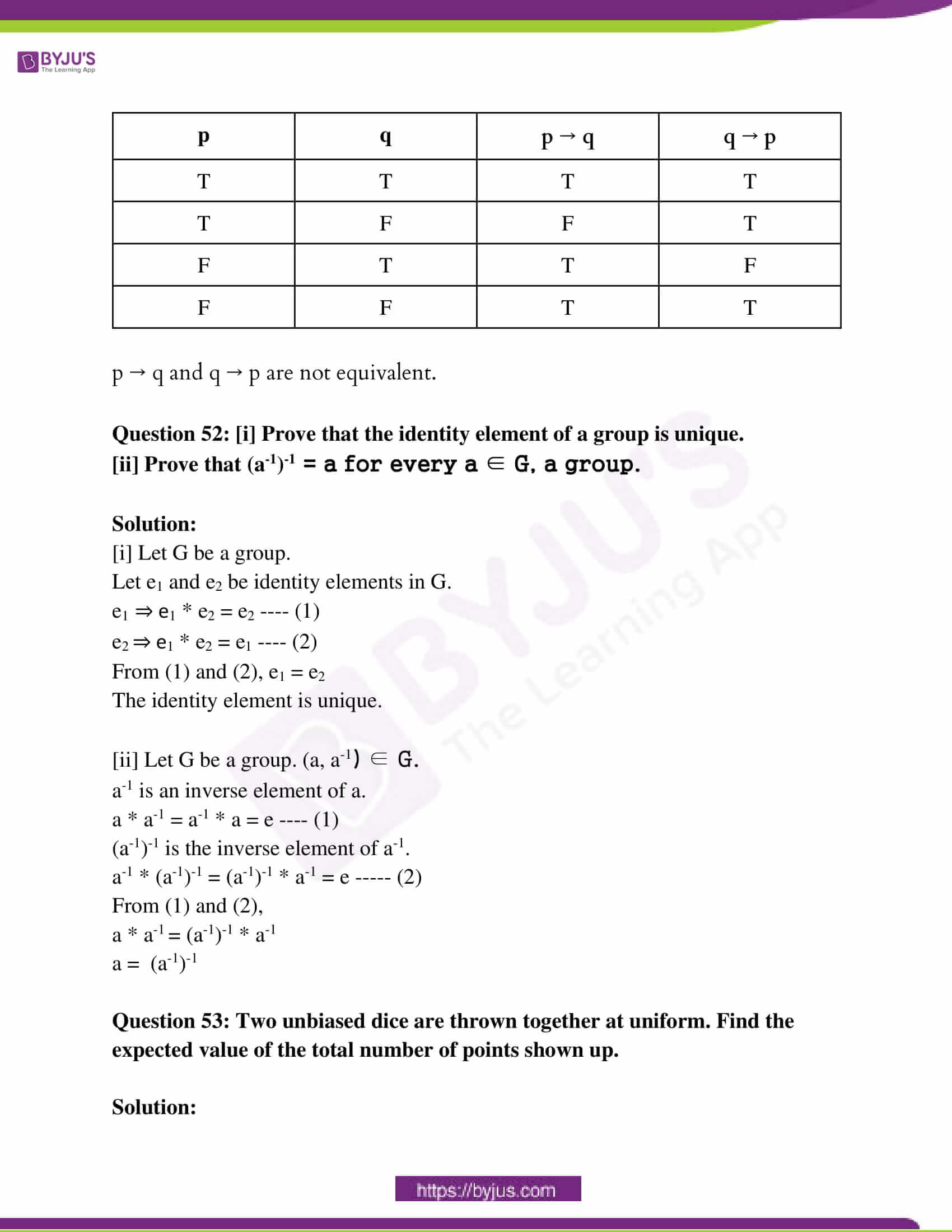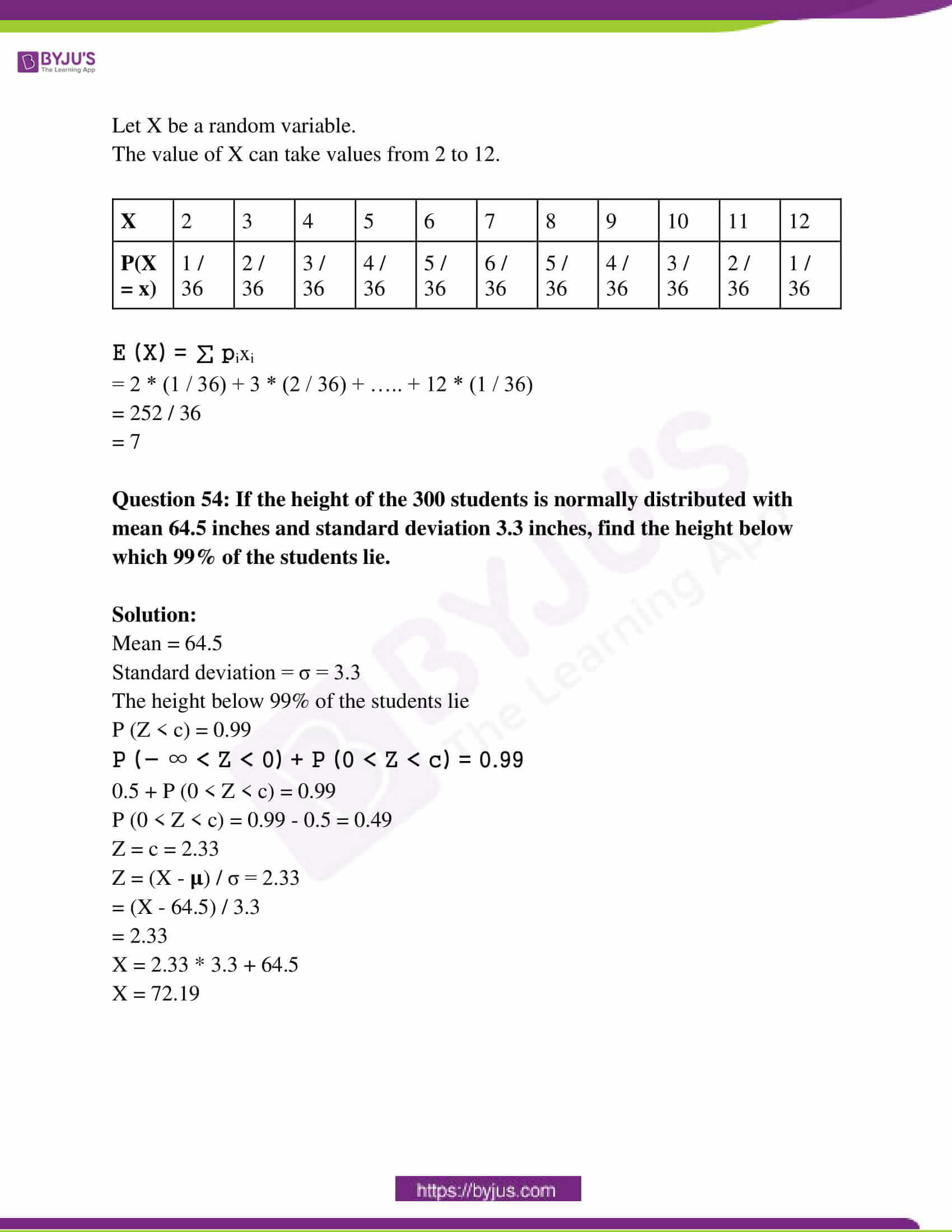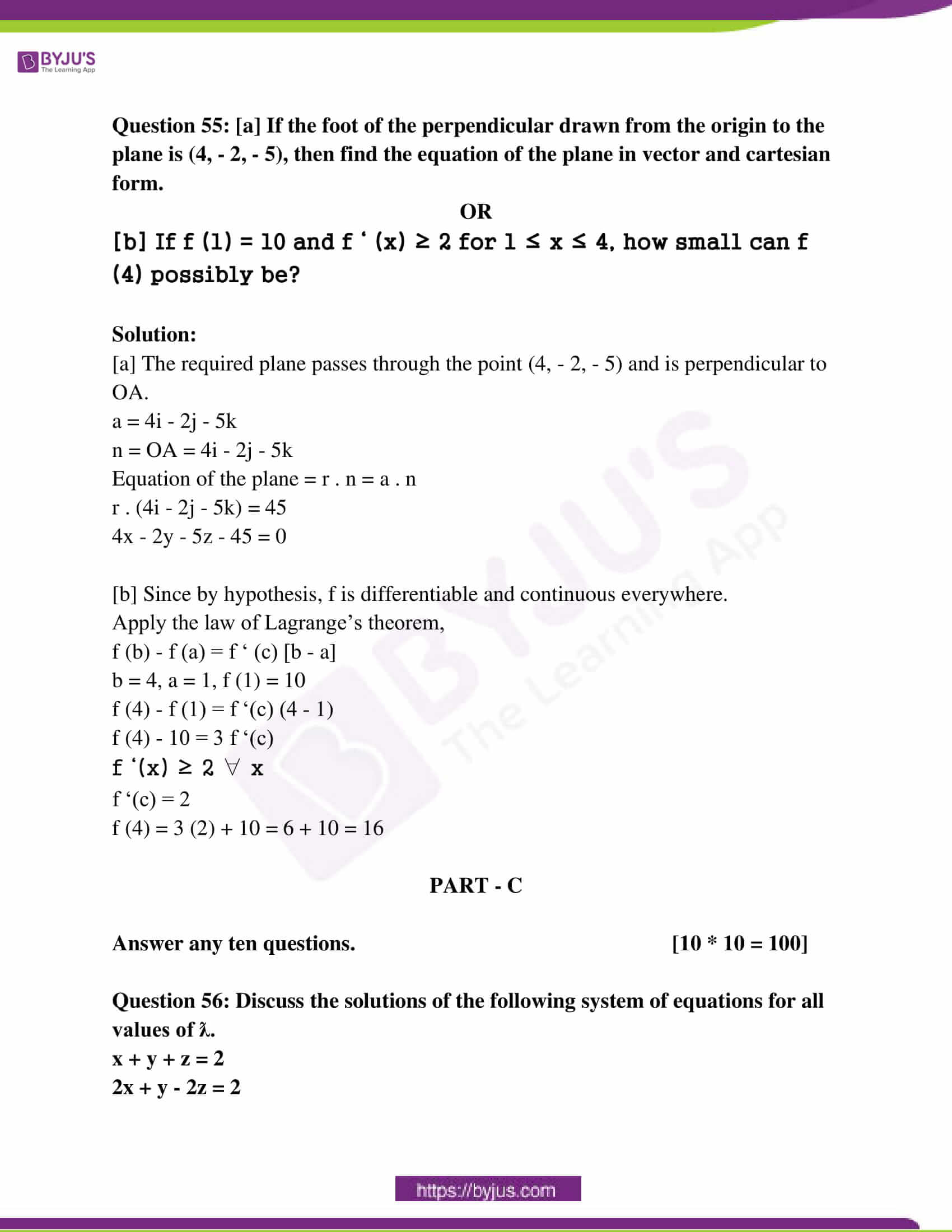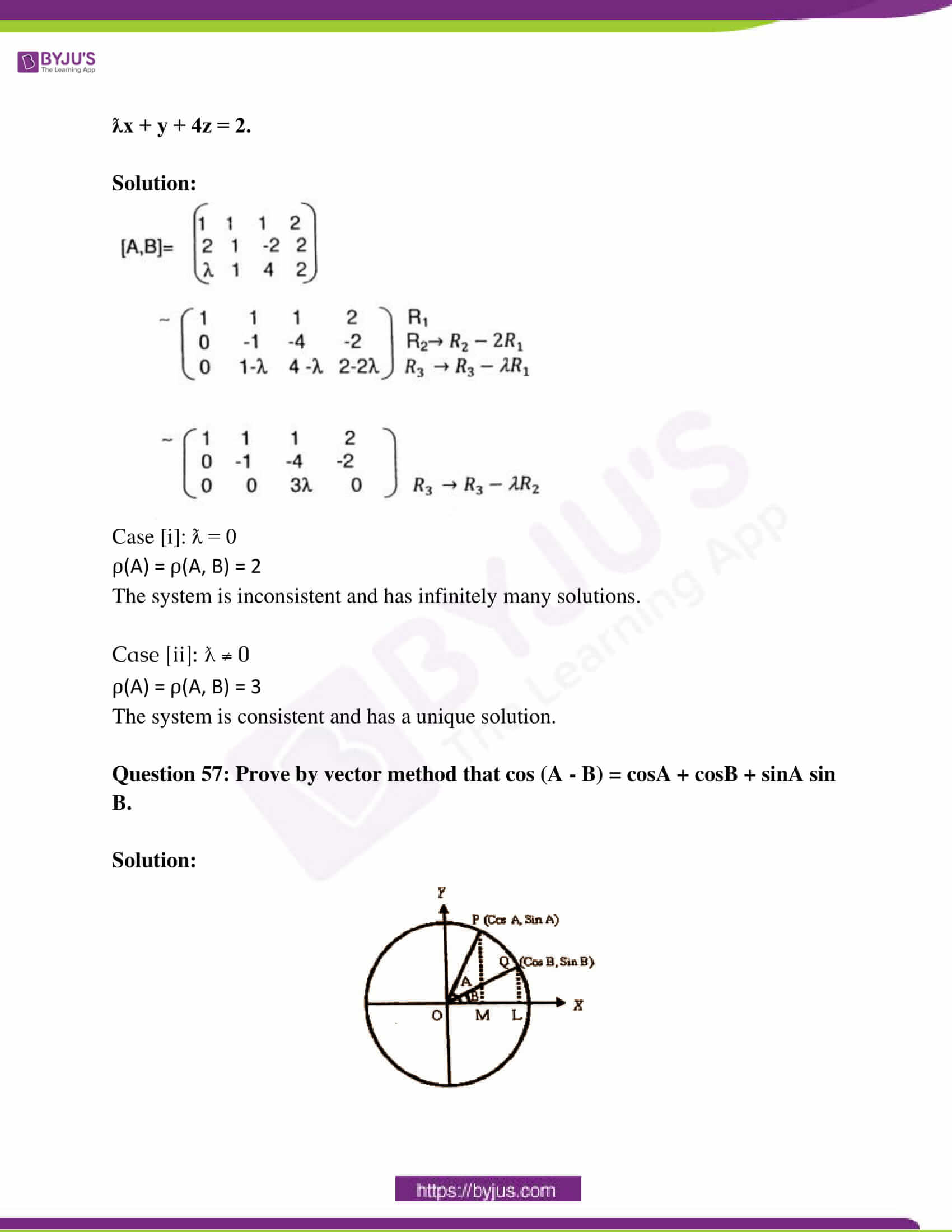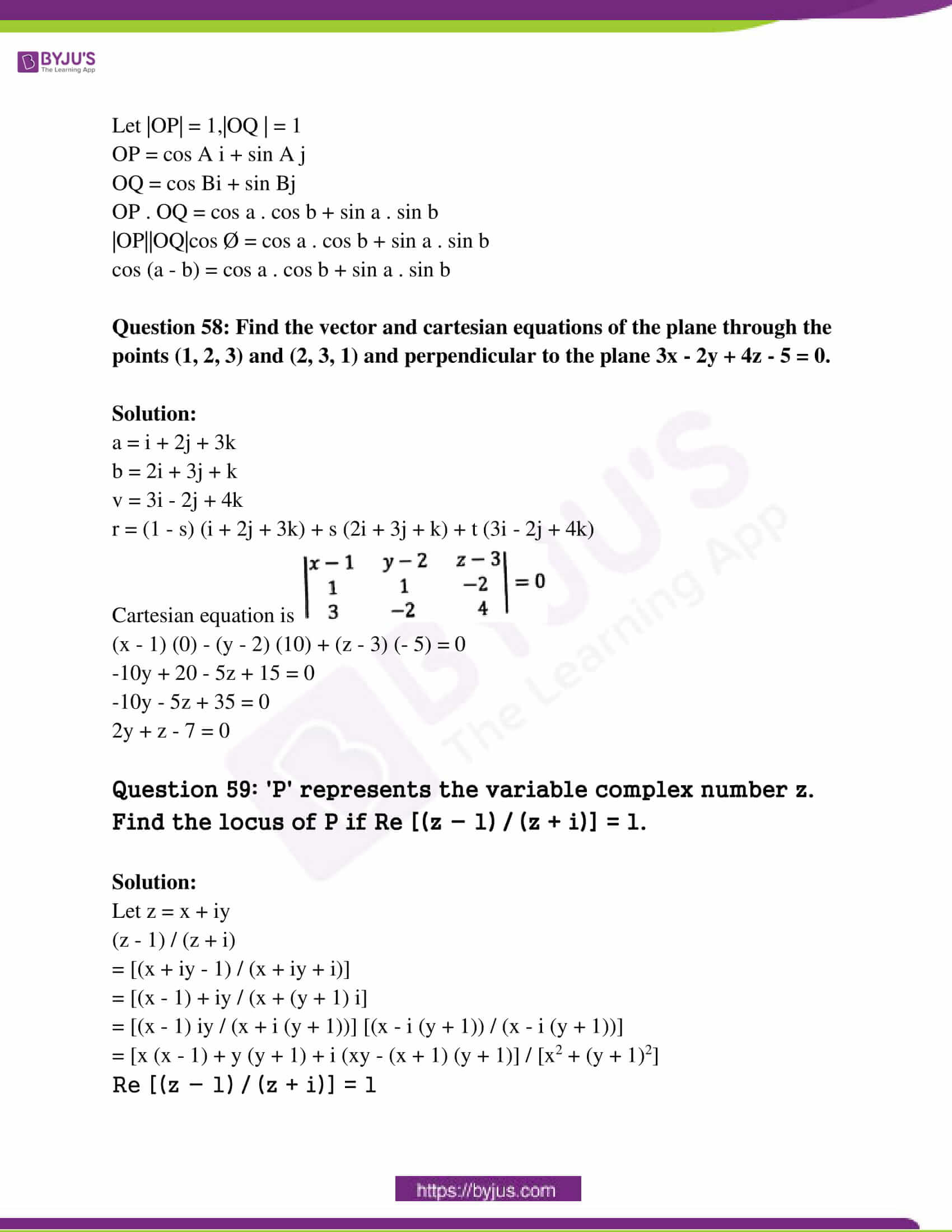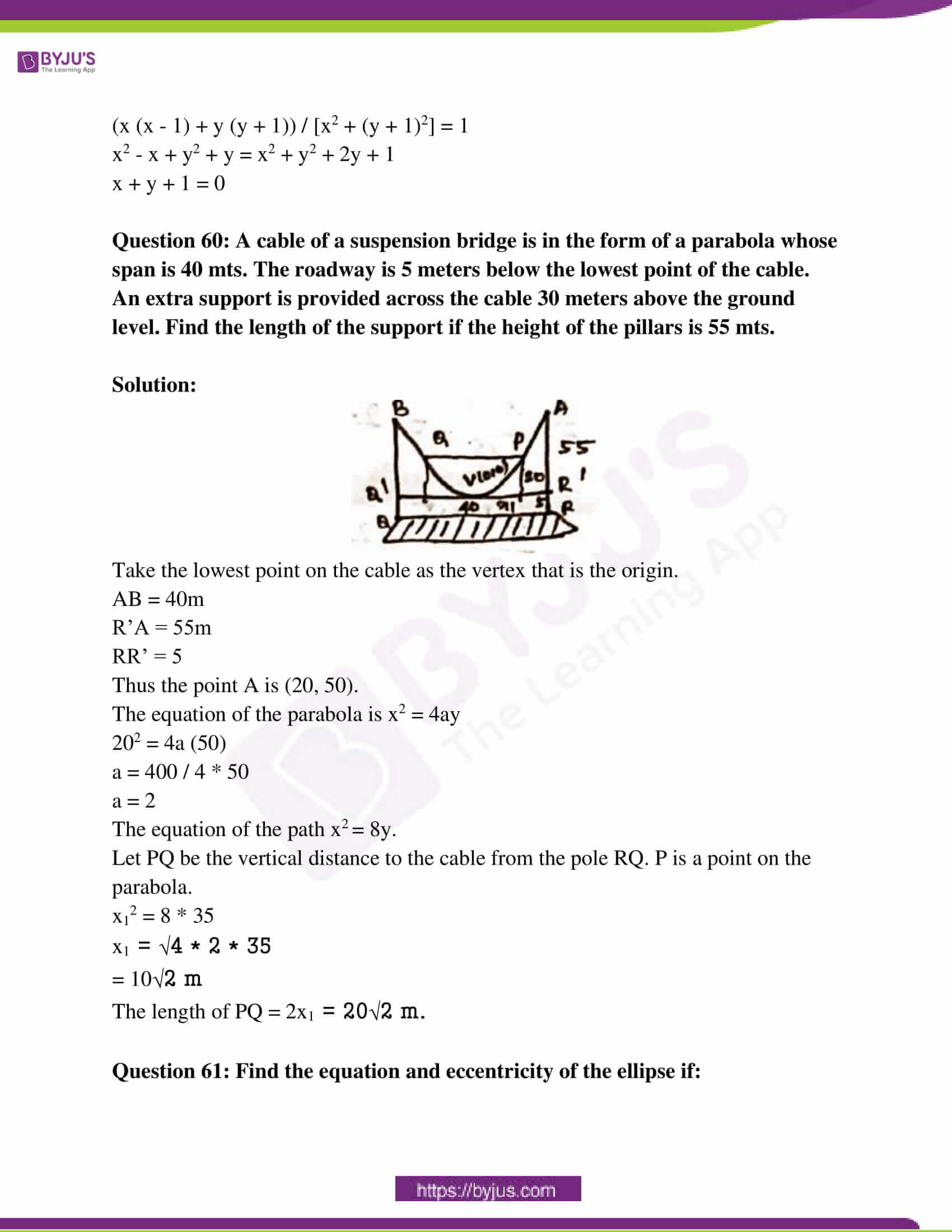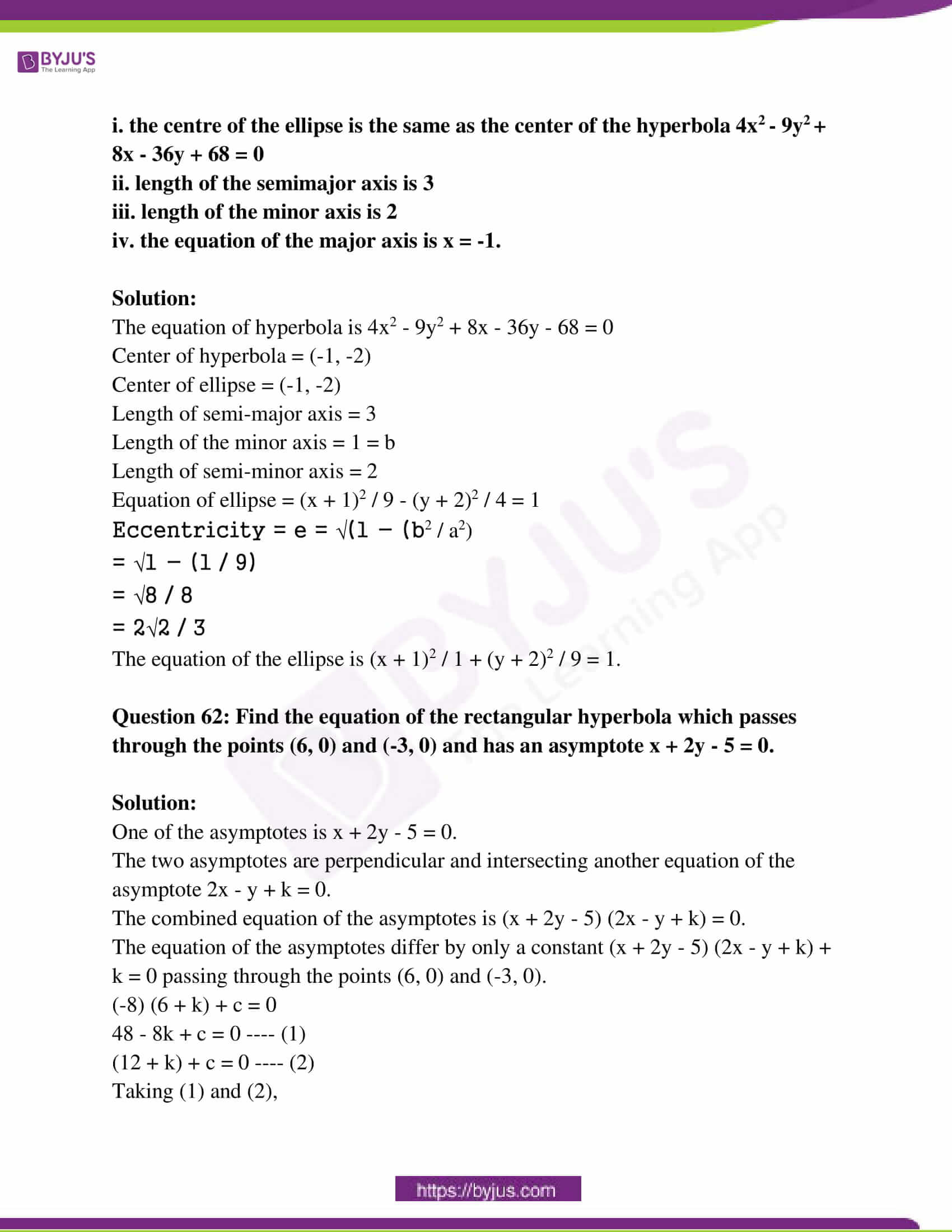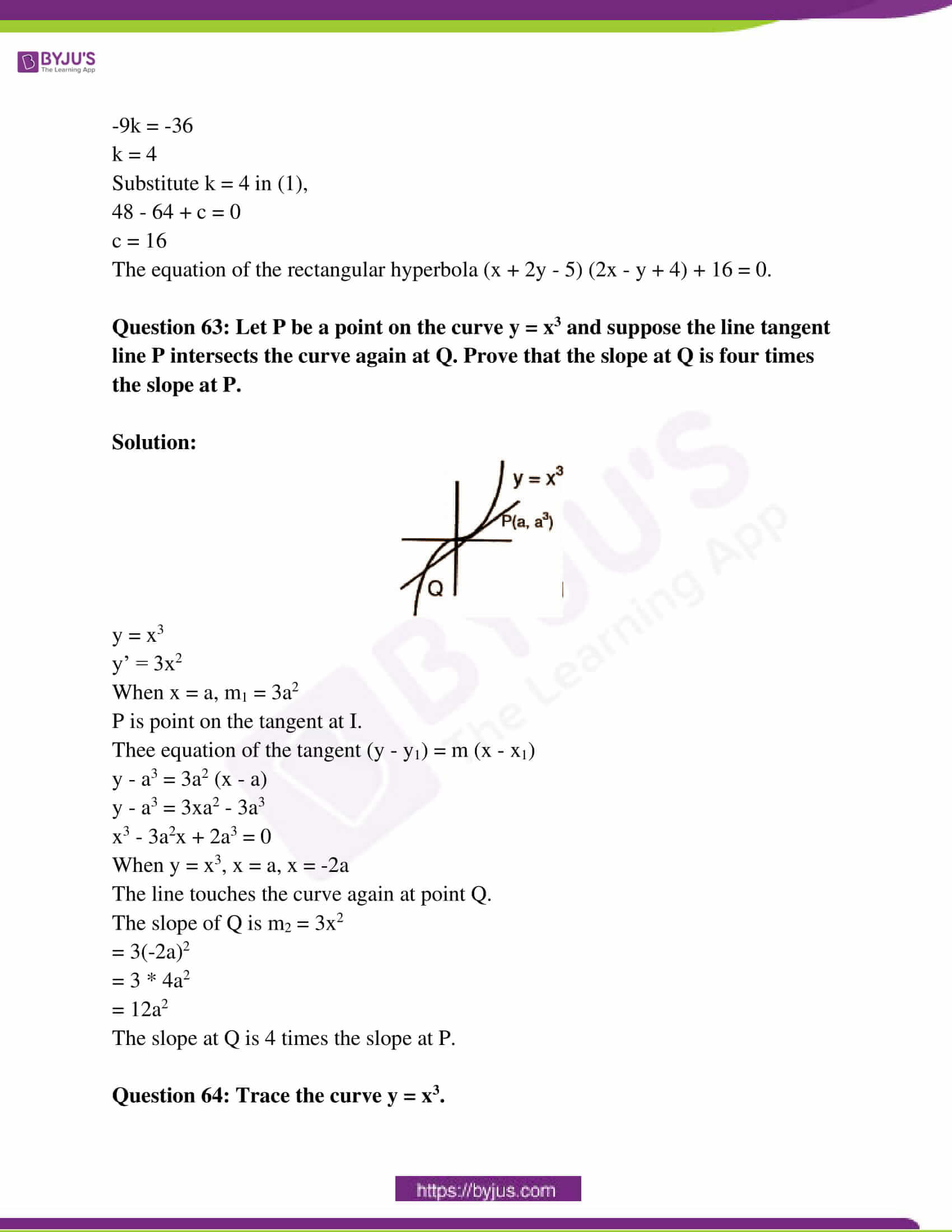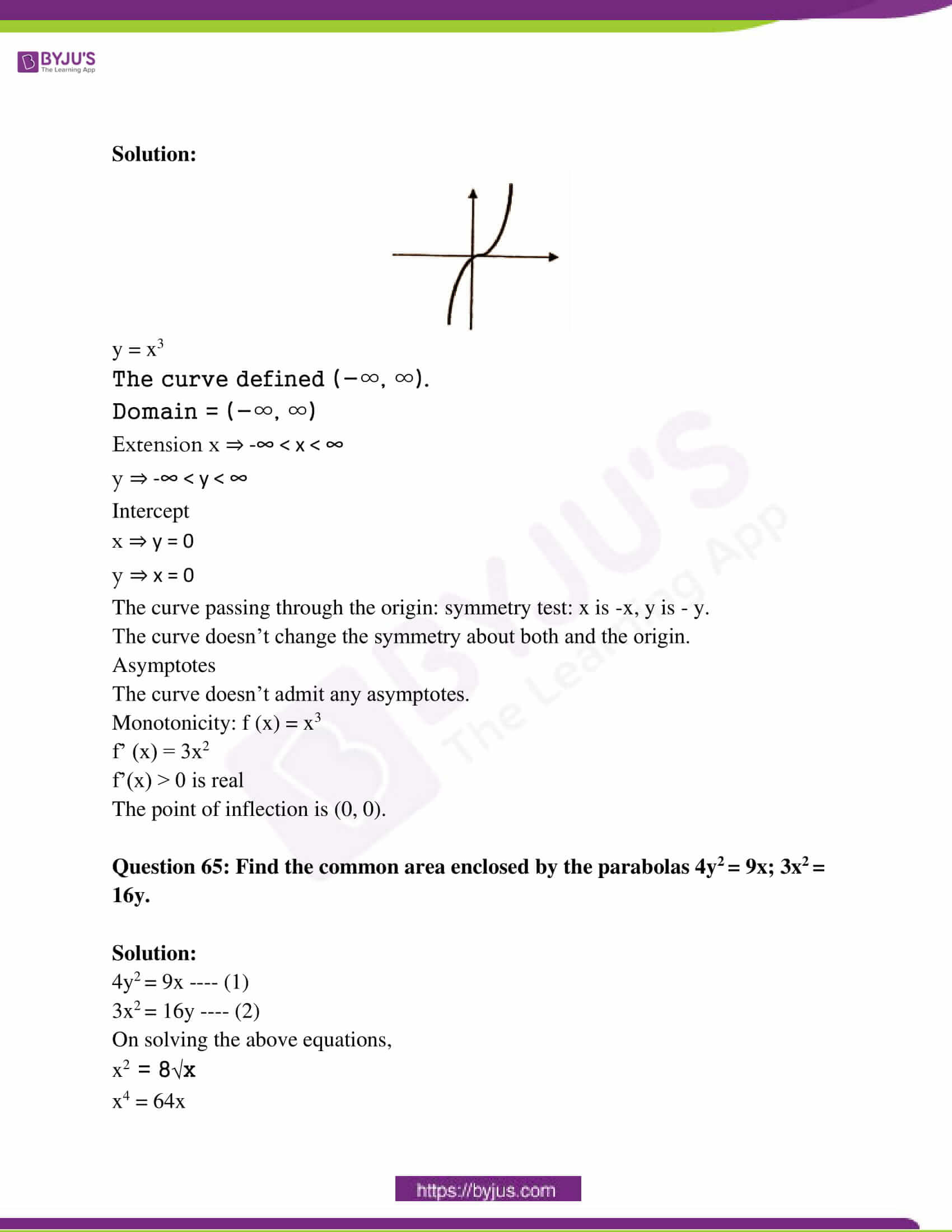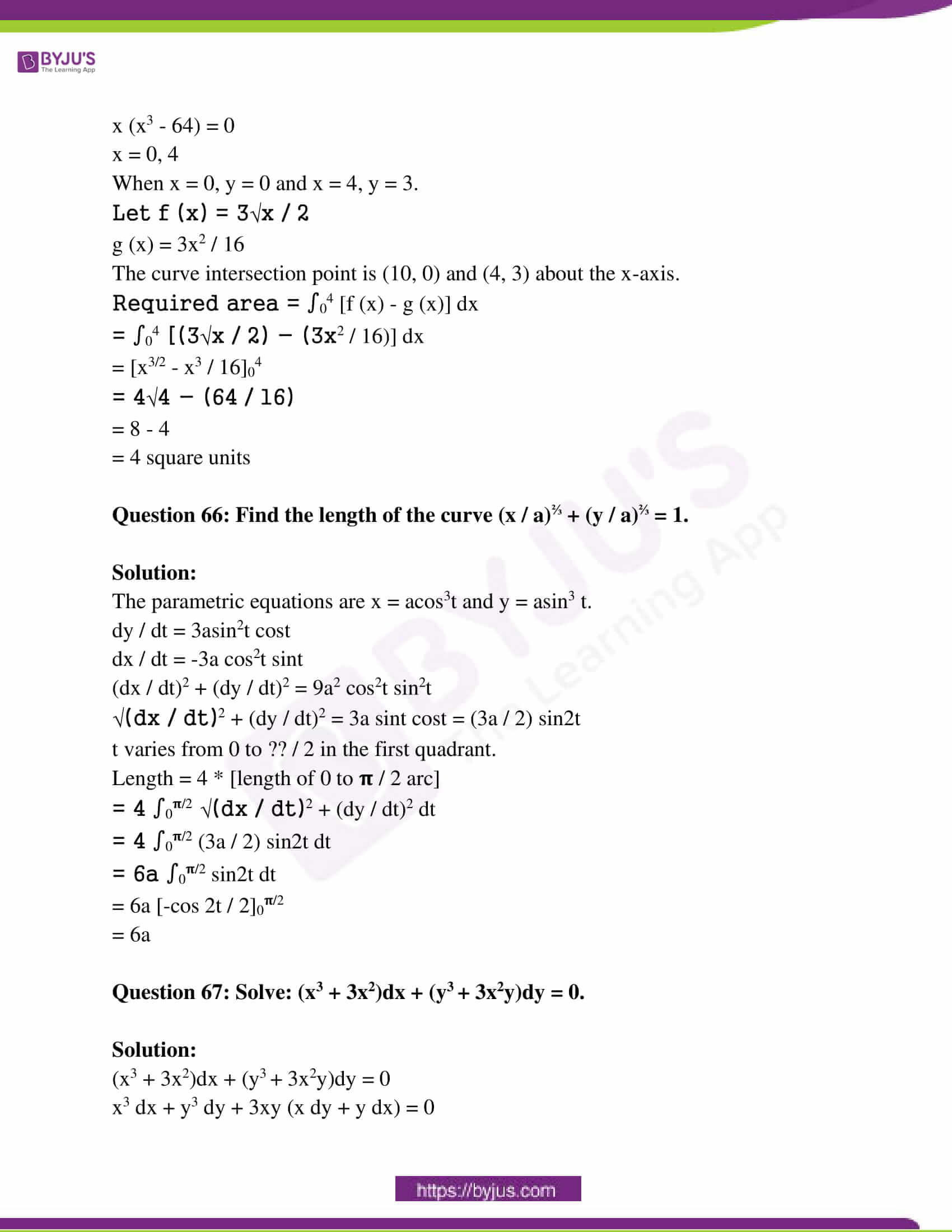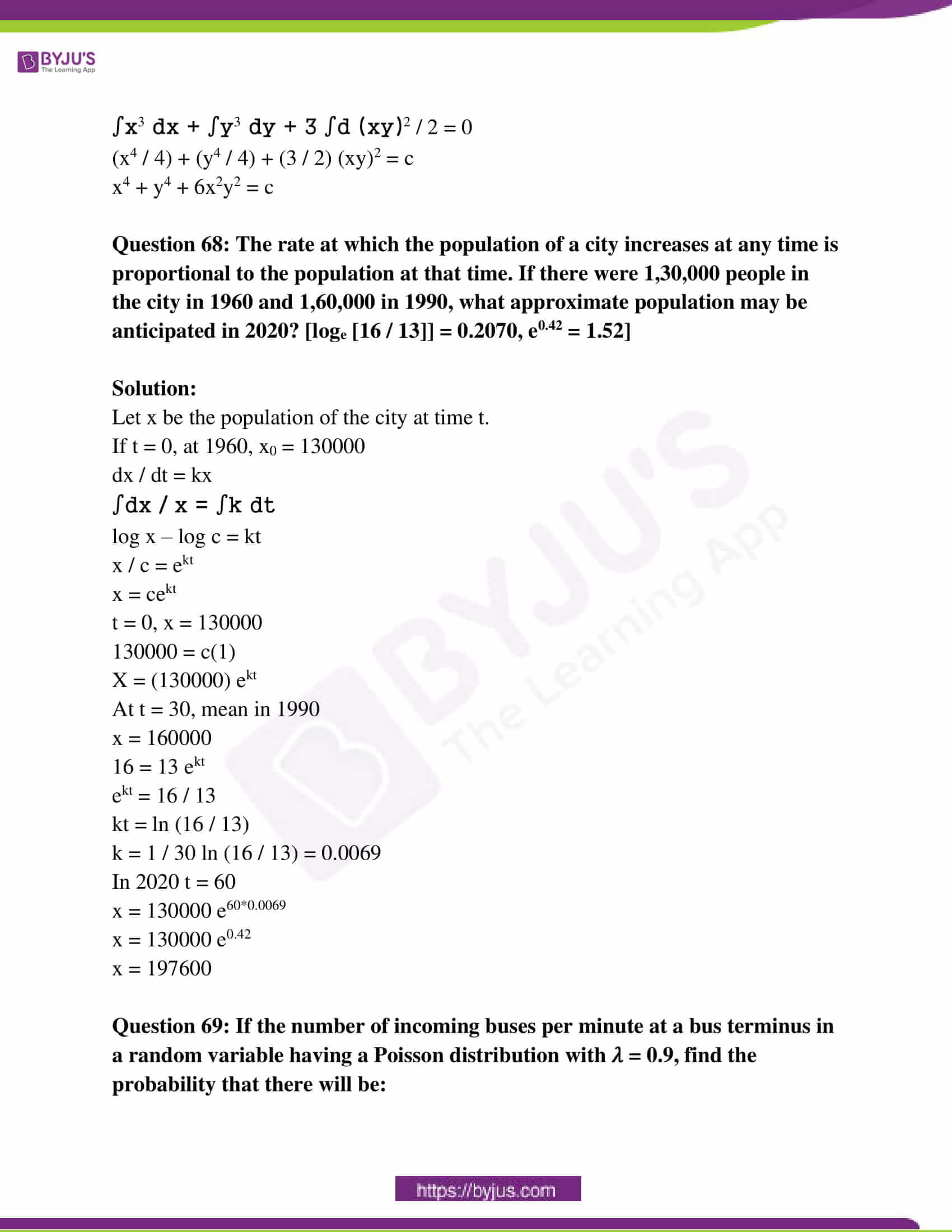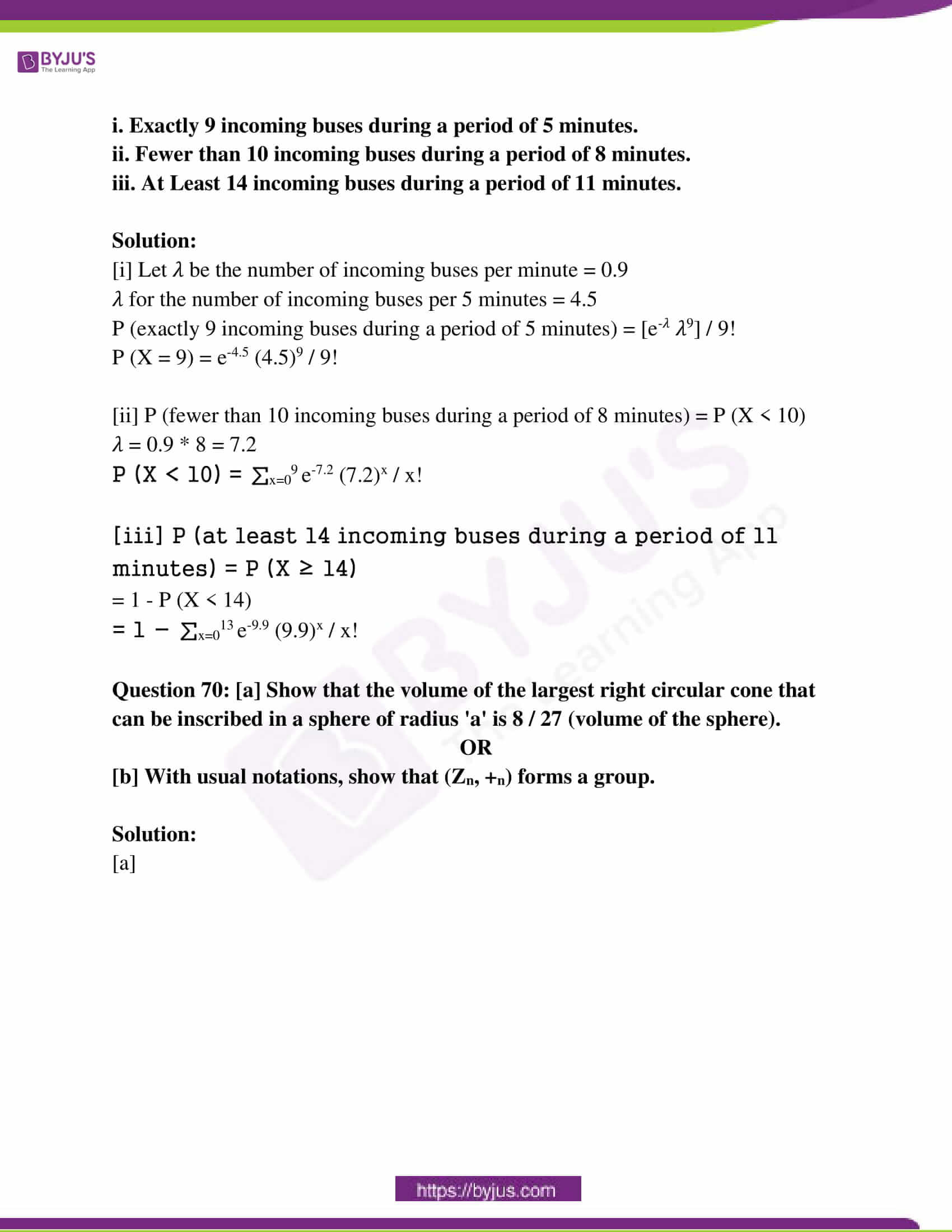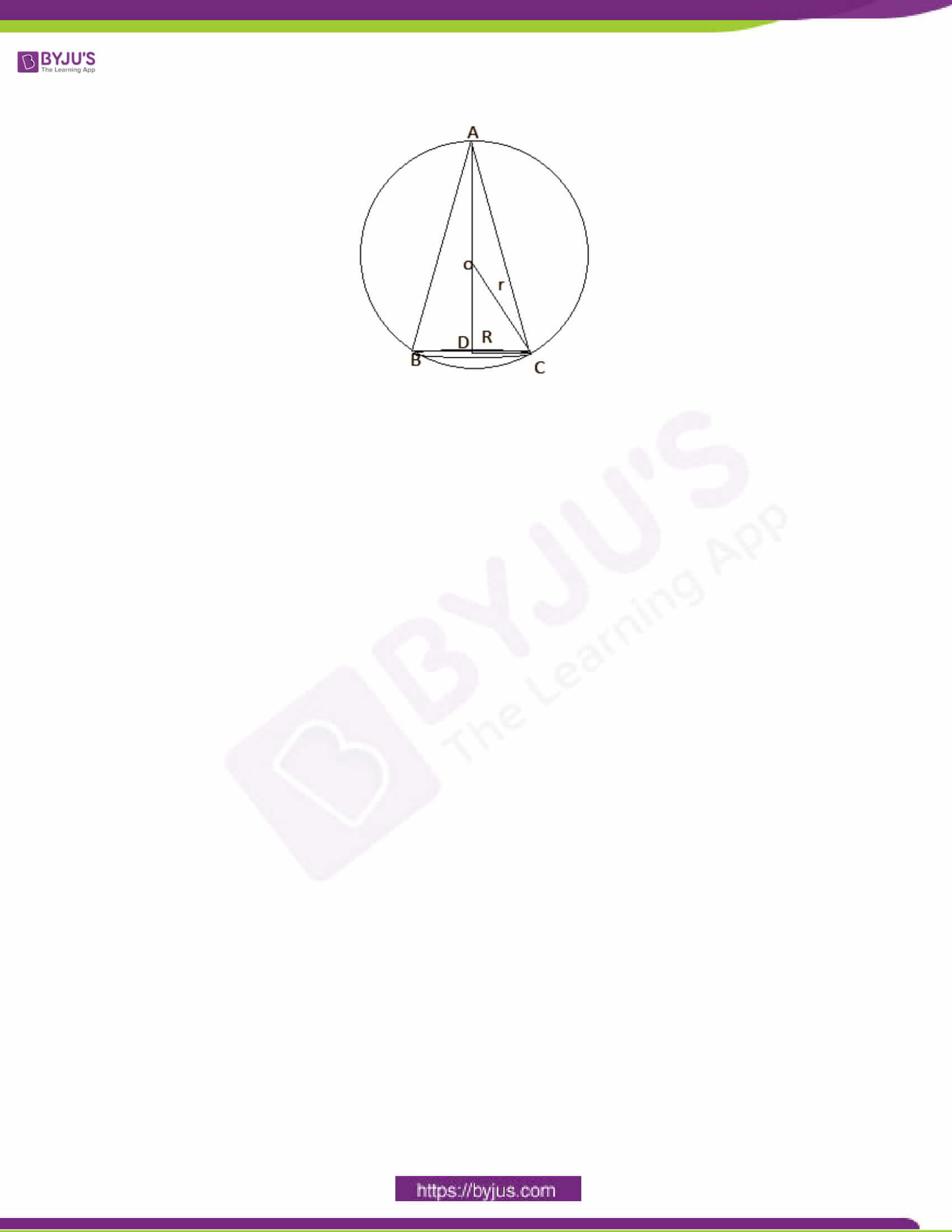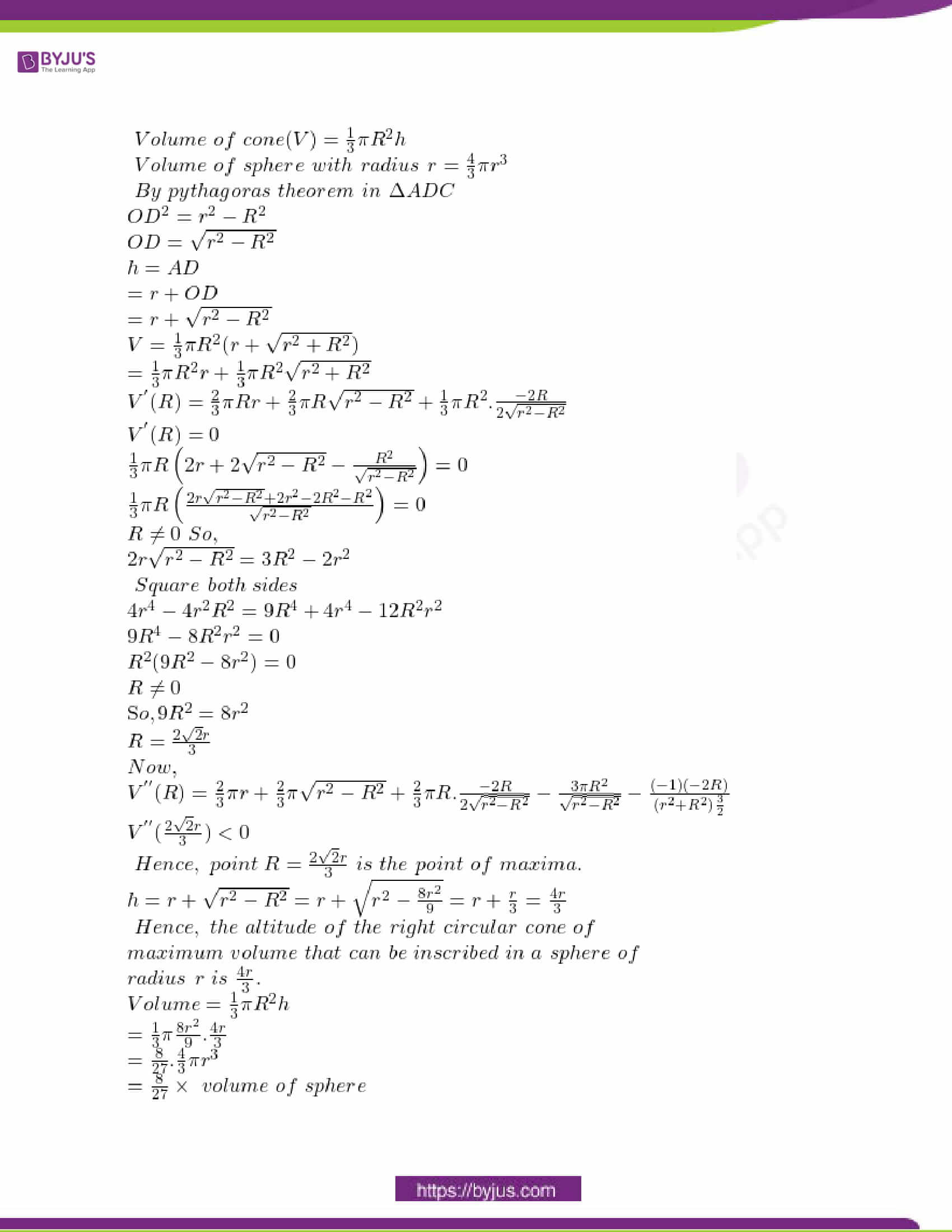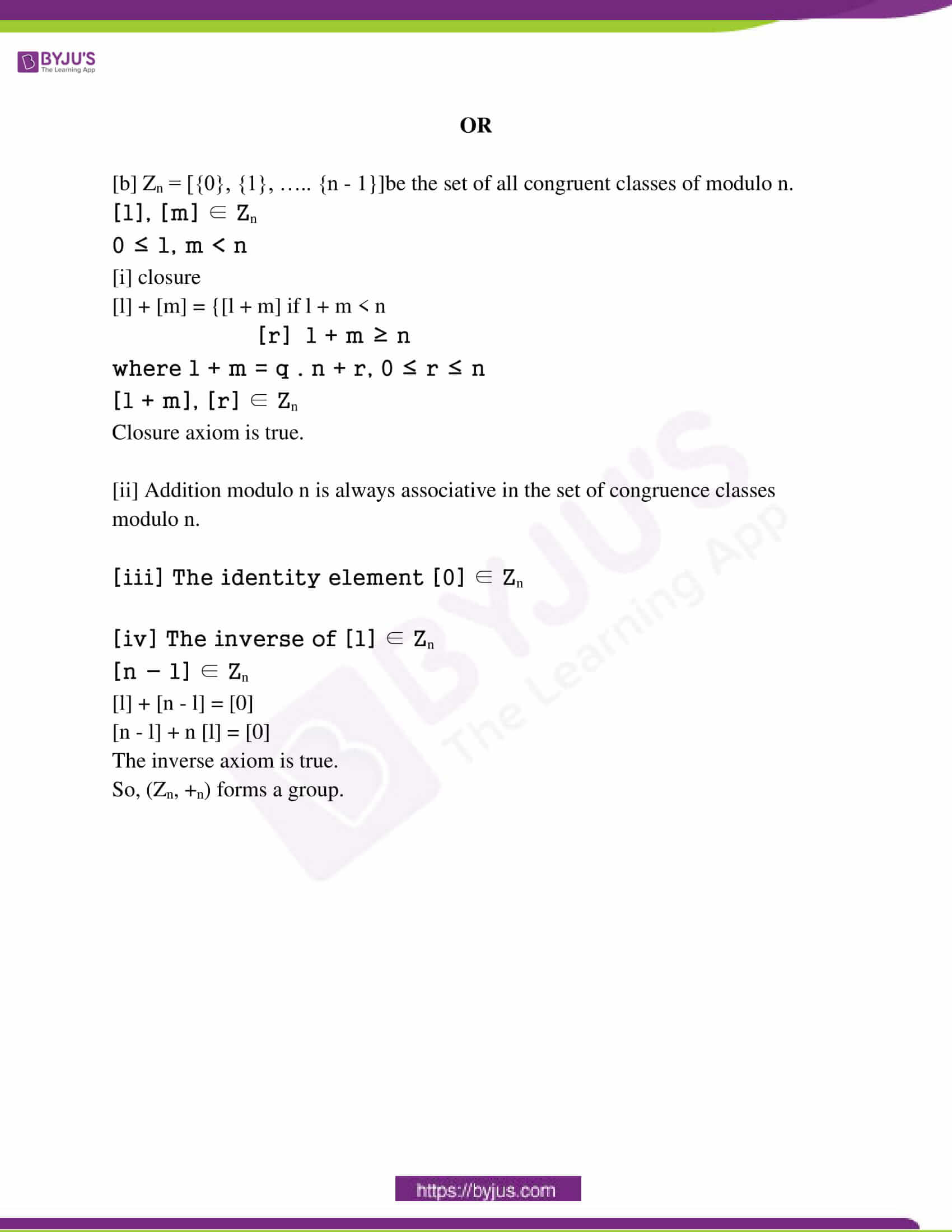PART – A

Choose the most appropriate answer from the given four alternatives and

write the option code and the corresponding answer. [40 * 1 = 40]

Question 1: The order and degree of the different equation y’ + y’’2 = (x + y’’)2 is

(a) 2, 1 (b) 1, 1 (c) 2, 2 (d) 1, 2

Solution: (a)

Question 2: The value of ‘a’ so that the curves y = 3ex and y = (a / 3) e-x intersect orthogonally is:

(a) 1 / 3 (b) – 1 (c) 3 (d) 1

Solution: (d)

y = 3ex

y = (a / 3) e-x

dy / dx = 3ex = m1

dy / dx = (- a / 3) ex = m2

m1 . m2 = -1

3ex . (a / 3) ex = -1

a = 1

Question 3: The value of ∫0𝛑 sin4x dx is

(a) 0 (b) 3𝛑 / 16 (c) 3𝛑 / 8 (d) 3 / 16

Solution: (c)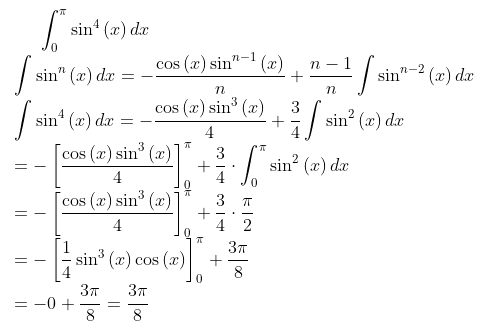Question 4: r = si + tj is the equation of:

(a) yoz plane

(b) a straight line joining the points i and j

(c) zox plane

(d) xoy plane

Solution: (d)

The line represents a straight line joining the point i, j which represents the x, y plane.

Question 5: If p is T and q is F, which of the following have the truth value T?

(i) p ˅ q (ii) ∼ p ˄ q (iii) p ˅ ∼ q (iv) p ˄ ∼ q

(a) (i), (iii), (iv) (b) (i), (ii), (iii) (c) (ii), (iii), (iv) (d) (i), (ii), (iv)

Solution: (a)

Question 6: In a system of 3 linear non-homogeneous equations with three unknowns, if ∆ = 0; and ∆x = 0; ∆y ≠ 0; ∆z = 0 then the system has:

(a) infinitely many solutions (b) unique solutions

(c) no solution (d) two solutions

Solution: (c)

By Cramer’s rule

X = Δx / x

Y = Δy / y

Z = Δz / z

So no solutions.

Question 7: If a × (b × c) + b × (c × a) + c × (a × b) = x × y then:

(a) x and y are parallel

(b) x = 0

(c) x = 0 or y = 0 or x and y are parallel

(d) y = 0

Solution: (c)

a x (b x c) + b x (c x a) + c x (a x b) = x * y

X x Y= 0

xy sin Ø â = 0

Ø = 0

X is parallel to y.

Question 8: The slope of the tangent to the curve y = 3x2 + 3 sinx at x = 0 is:

(a) 1 (b) 3 (c) -1 (d) 2

Solution: (b)

y = 3x2 + 3sinx

dy / dx = 6x + 3 cosx

At x = 0

dy / dx = 3

Question 9: The differential of y if y = √x4 + x2 + 1 is:

(a) 1 / 2 (4x3 + 2x)−1/2

(b) 1 / 2 (4x3 + 2x)−1/2 dx

(c) 1 / 2 (x4 + x2 + 1)−1/2 (4x3 + 2x)

(d) 1 / 2 (x4 + x2 + 1)−1/2 (4x3 + 2x) dx

Solution: (d)

y = (x4 + x2 + 1)½

dy / dx = (1 / 2y) . 2 (2x3 + x)

dy / dx = (1 / 2) (x4 + x2 + 1)−1/2. (4x3 + 2x)

Question 10: If cosx is an integrating factor of the differential equation dy / dx + Py = Q then P =

(a) tanx

(b) − cotx

(c) – tanx

(d) cotx

Solution: (c)

e∫P dx = cosx

∫P dx = ln . cosx

P = (1 / cosx) . – sinx

P = – tanx

Question 11: The differential equation of all circles with center with center at the origin is:

(a) x dx + y dy = 0 (b) x dy + y dx = 0

(c) x dx – y dy = 0 (d) x dy – y dx = 0

Solution: (a)

The equation of the circle is given by

x2 + y2 = a2

2x dx + 2y dy = 0

x dx + y dy = 0

Question 12: If |z − z1| = |z − z2| then the locus of z is:

(a) a straight line passing through the origin

(b) a circle with centre at the origin

(c) is a perpendicular bisector of the line joining z1 and z2

(d) a circle with centre at z1

Solution: (c)

|z – z1| = |z – z2|

Let z1 = x1 + iy1 and z2 = x2 + iy2

z – z1 = (x – x1) + i (y – y1)

z – z2 = (x – x2) + i (y – y2)

Equate the above equations,

(x22 – x12) + (y22 – y12) = 2x (x2 – x1) + 2y (y2 – y1)

Question 13: If a line makes 45°, 60° with positive direction of axes x and y then the angle it makes with the z-axis is:

(a) 45° (b) 30° (c) 60° (d) 90°

Solution: (c)

l2 + m2 + n2 = 1

cos2 (45) + cos2 (60) + cos2 x = 1

cos2 x = 1 / 4

x = 60o

Question 14: The curve y2 (x − 2) = x2 (1 + x) has:

(a) asymptotes parallel to both axes (b) an asymptote parallel to x-axis

(c) no asymptotes (d) an asymptote parallel to y-axis

Solution: (d)

y2 (x − 2) = x2 (1 + x)

y = (x2 (1 + x) / (x – 2))1/2

For vertical asymptote, x – 2 = 0

x = 2

Question 15: Which of the following statements is correct regarding the homogeneous system?

(a) has only non-trivial solutions

(b) always inconsistent

(c) has only a trivial solution only if the rank of the coefficient matrix is equal to the number of unknowns

(d) has only a trivial solution

Solution: (c)

It has a trivial solution if the rank of the coefficient matrix is equal to the number of unknowns.

Question 16: The value of ‘c’ of Lagrange Mean value theorem for f (x) = √x, when a = 1 and b = 4 is:

(a) 1 / 2 (b) 9 / 4 (c) 1 / 4 (d) 3 / 2

Solution: (b)

F (x) = √x

F ’ (c) = [f (b) – f (a)] / [b – a]

(1 / 2) (c)1/2 = f (4) – f (1) / 3

1 / c = 4 / 9

C = 9 / 4

Question 17: The point of intersection of tangents at t1 = t and t2 = 3t to the parabola y2 = 8x is:

(a) (t2, 4t) (b) (6t2, 8t) (c) (4t, t2) (d) (8t, 6t2)

Solution: (b)

y2 = 8x

(2y) dy / dx = 8x

dy / dx = 4 / y

m1 = 1 / t

m2 = 1 / 3t

Equation of lines

y = x + 2t2 / t

(y – 12t) / (x – 18t2) = 1 / 3t

(x + 2t2) / (t2 – 12t2) = 1 / 3t

3x – 30t2 = x – 18t2

x = 6t2, y = 8t

Question 18: If 𝜔 is a cube root of unity then the value of (1 − 𝜔 + 𝜔2)4 + (1 + 𝜔 − 𝜔2)4 is:

(a) – 16 (b) 0 (c) -30 (d) 32

Solution: (a)

(- 2w)4 + (- 2w2)4

24 .w4 + 24 . w8

= 24 (w + w2)

= – 24

= – 16

Question 19: Which of the following is not true?

(a) If the last column of its truth table contains only F then it is a contradiction

(b) The negation of a negation of a statement is the statement itself

(c) If p and q are two statements then p ↔ q is a tautology

(d) If the last column if its truth table contains only T then it is a tautology

Solution: (c)

Question 20: The volume of solid generated by rotating the triangle with vertices at (0, 0), (3, 0) and (3, 3) about x-axis is:

(a) 36𝜋 (b) 18𝜋 (c) 9𝜋 (d) 2𝜋

Solution: (c)

Volume generated = volume of cone = (1 / 3) 𝜋r2h

= (1 / 3) (22 / 7) (32) 3

= 9𝜋

Question 21: In the set of integers under the operation ∗ defined by a ∗ b – a + b – 1, the identity element is:

(a) a (b) 0 (c) b (d) 1

Solution: (d)

Let e be the identity element.

a * e = a + e – 1

a = a + e – 1

e =1

e * a = e + a – 1

a = e + a – 1

e = 1

Question 22: The modulus and amplitude of the complex number [e3−i (𝜋/4)]3 are respectively:

(a) e6, −3𝜋 / 4 (b) e9, 𝜋 / 2

(c) e9, −3𝜋 / 4 (d) e9 , −𝜋 / 2

Solution: (c)

Z = e9 . e (-3𝜋/4)i

= e9 [cos (- 3𝜋 / 4) + i sin (- 3𝜋 / 4)]

|Z| = e9

Amplitude (z) = – 3𝜋 / 4

Question 23: The locus of the foot of perpendicular from the focus on any tangent to the hyperbola x2 / a2 − y2 / b2 = 1 is:

(a) x2 + y2 = a2 + b2 (b) x2 + y2 = a2 – b2

(c) x = 0 (d) x2 + y2 = a2

Solution: (d)

Question 24: The surface area of the solid obtained by revolving the region bounded by y = 2x, x = 0 and x = 2 about x-axis, is:

(a) √5𝜋 (b) 8√5𝜋 (c) 4√5𝜋 (d) 2√5𝜋

Solution: (b)

Surface area = 𝜋r (h2 + r2)1/2

= 𝜋 4 [22 + 42]1/2

= 8√5𝜋

Question 25: A random variable X has the following probability mass function:

 X – 2 3 1 P (X = x) ƛ / 6 ƛ / 4 ƛ / 12

Then the value of ƛ is:

(a) 3 (b) 1 (c) 4 (d) 2

Solution: (d)

λ / 6 + λ / 4 + λ / 12 = 1

λ = 2

Question 26: If, in a Poisson distribution P (X = 0) = k then the variance is:

(a) e𝜆 (b) log (1 / k) (c) 1 / k (d) log k

Solution: (b)

In poisson distribution,

P (x = r) = e . [λr / r!]

For r = 0,

P (x = 0) = e

P (x = 0) = k

e = k

λ = ln (1 / k)

Question 27: ∫0 x6 e−x/2 dx =

(a) 26⌊6 (b) ⌊6 27 (c) 27⌊6 (d) ⌊6 26

Solution: (c)

After integration I = 2 x 6 ∫0 e-x/2 x6

I = 27 . 6!

Question 28: If the point is represented by the complex number iz is rotated about the origin through an angle 𝜋 / 2 in the counterclockwise direction then the complex number representing the new position is:

(a) –z (b) iz (c) z (d) -iz

Solution: (a)

Let z = e and i𝜋 is rotated about 𝜋 / 2 angle.

So z1 = ie(i(𝜋 / 2 + Ø))

= i [cos(𝜋 / 2 + Ø) + 𝑖 sin (𝜋 / 2 + Ø)]

= i [- sin Ø + i cos Ø]

= – [cos Ø = i sin Ø]

= – z

Question 29: Inverse of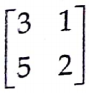is: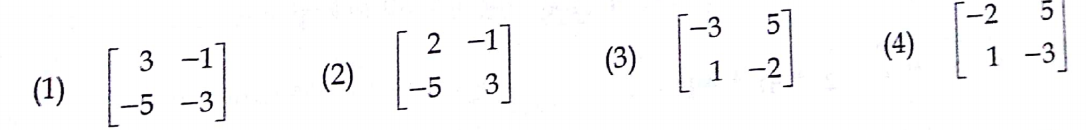Solution: (2)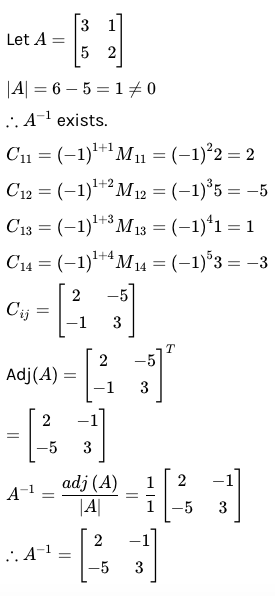Question 30: The mean of a binomial distribution is 5 and its standard deviation is 2. Then the value of n and p are:

(a) (1 / 5 , 25) (b) (4 / 5 , 25) (c) (25, 1 / 5) (d) (25, 4 / 5)

Solution: (c)

Mean = np = 5

S.D = √npq

2 = √np (1 – p)

np (1 – p) = 4

5 (1 – p) = 4

p = 1 / 5

n = 25

Question 31: The vector equation of a plane passing through the line of intersection of the planes r . n1 = q1 and r . n2 = q2 is:

(a) 𝑟 × 𝑛1 + 𝑟 × 𝑛2 = 𝑞1 + 𝑞2

(b) (𝑟 . 𝑛1 − 𝑞1) + 𝜆 (𝑟 . 𝑛2 − 𝑞2) = 0

(c) 𝑟 × 𝑛1 − 𝑟 × 𝑛2 = 𝑞1 + 𝑞2

(d) 𝑟 . 𝑛1 + 𝑟 . 𝑛2 = 𝑞1 + 𝜆𝑞2

Solution: (b)

The plane passing through the intersection of p1 and p2 is (𝑟 . 𝑛1 − 𝑞1) + 𝜆 (𝑟 . 𝑛2 − 𝑞2) = 0.

Question 32: If dy / dx = x – y / x + y then:

(a) 𝑥2 + 𝑦2 − 2𝑥y = 𝑐

(b) 2𝑥y + 𝑦2 + 𝑥2 = 𝑐

(c) 𝑥2 − 𝑦2 − 2𝑥y = 𝑐

(d) 𝑥2 + 𝑦2 − 𝑥 + 𝑦 = 𝑐

Solution: (c)

dy / dx = (x – y) / (x + y)

Let y = vx

dy / dx = v + x (dv / dx)

V + x (dv / dx) = (1 – v) / (1 + v)

x (dv / dx) = (1 – v – v – v2) / (1 + v)

∫(1 + v) / (1 – 2v – v2) dv = ∫dx / x

1 – 2v – v2 = t ∫ dt / – 2t =ln x + ln c

ln (1 – 2y / x – y2 / x2) = ln (x-2) . c

x2 – 2xy – y2 = c

Question 33: If a is non-zero vector and m is a non-zero scalar then ma is unit vector if:

(a) a = 1 / |m| (b) m = ± 1 (c) a = 1 (d) a = |m|

Solution: (a)

|ma| = 1

|m| a = 1

a = 1 / |m|

Question 34: Which of the following is not true regarding the normal distribution?

(a) the point of inflexion is at X = 𝜇 ± 𝜎

(b) skewness is zero

(c) maximum height of the curve is 1 / √2𝜋

(d) mean = median = mode

Solution: (c)

Question 35: If A is a scalar matrix with scalar k ≠ 0, of order 3, then A−1 is:

(a) (1 / k) I (b) (1 / k2) I (c) kI (d) (1 / k3) I

Solution: (a)

Question 36: Which of the following is not a group?

(a) (Z, .) (b) (Zn, +n) (c) (R, +) (d) (Z, +)

Solution: (a)

Question 37: The statement: “If f has a local extremum (minimum or maximum) at c and if f ’(c) exists then f ’(c) = 0” is:

(a) Law of mean

(b) The extreme value theorem

(c) Rolle’s theorem

(d) Fermat’s theorem

Solution: (d)

Question 38: The coordinate of the vertices of the rectangular hyperbola xy=16 are:

(a) (4, 0), (-4, 0) (b) (4, 4), (-4, -4)

(c) (8, 0), (-8, 0) (d) (2, 8), (-2, -8)

Solution: (b)

Question 39: The sum of the distance of any point on the ellipse 4x2 + 9y2 = 36 from (√5, 0) and (- √5, 0) is:

(a) 6 (b) 4 (c) 18 (d) 8

Solution: (a)

Let p (√5, 0) and q (- √5, 0)

Sum of the distance of any point on the ellipse from the focus (± √5, 0).

Sum of major axis = 2 * 3 = 6

Question 40: The point of intersection of the lines r = (−i + 2j + 3k) + t (−2i + j + k) and r = (2i + 3j + 5k) + s (i + 2j + 3k) is:

(a) (1, 1, 2) (b) (2, 1, 1) (c) (1, 1, 1) (d) (1, 2, 1)

Solution: (a)

From equating the points,

– 2t – 1 = s + 2

s = 2t = -3 (i)

t + 2 = 2s + 3

2s – t = -1 (ii)

3s + 5 = t + 3

3s – t = – 2 (iii)

From (i) and (ii),

t = -1 and s = -1

From equation (i), 3 (- 1) + 1 = – 2 = RHS

The points are (s + 2, 2s + 3, 3s + 5).

The point of intersection is (1, 1, 2).

PART – B

Answer any ten questions. [10 * 6 = 60]

Question 41: Find the adjoint of the matrix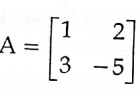and verify the result A (adj A) = (adj A) A = |A| I.

Solution: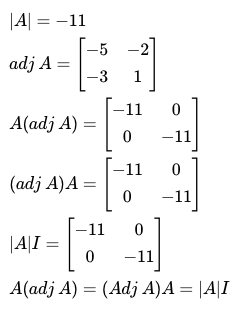Question 42: Solve the following non-homogeneous equations by using the determinant method.

2x + 2y + z = 5

x – y + z = 1

3x + y + 2z = 4

Solution:

AX = B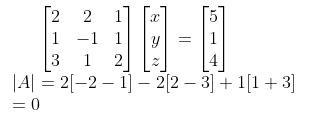The value of the determinant is 0.

The system is inconsistent with no solutions.

Question 43: [i] Show that the points whose position vectors are 4i – 3j + k, 2i – 4j + 5k, i – j form a right-angled triangle.

[ii] If A (-1, 4, -3) is one end of a diameter AB of the sphere x2 + y2 + z2 – 3x – 2y + 2z – 15 = 0, then find the coordinate of B.

Solution:

[i] a = 4i – 3j + k

b = 2i – 4j + 5k

c = i – j

a, b, c are position vectors and they form a triangle.

AB = OB – OA = b – a = – 2i – j + 4k

BC = OC – OB = c – b = – i + 3j – 5k

CA = OA – OC = a – c = 3i – 2j + k

AB + BC + CA = 0

AB . CA = (- 2i – j + 4k) . (3i – 2j + k) = 0

AB is perpendicular to CA.

AB, BC, CA form a right triangle.

[ii] Centre (-u, -v, -w) = (3 / 2, 1, -1)

The midpoint of AB is the centre

(3 / 2, 1, -1) = [(1 + x2) / 2, (4 + y2) / 2, (- 3 + z2) / 2]

x2 = 4, y2 = – 2, z2 = 1

The coordinate of B is (4, – 2, 1).

Question 44: Show that for any polynomial equation P(x) = 0, with real coefficients, imaginary roots occur in conjugate pairs.

Solution:

P (x) is a polynomial equation.

x = 0 is a root of the polynomial.

P (x) = xn an + xn-1 an-1 + xn-2 an-2 + …… + x0a0 = 0

Z is a root of a polynomial P (Z) = 0.

To prove that P (bar Z) = 0

P (bar Z) = (bar) [anzn + an-1zn-1 + …… + a0 = 0]

bar (anzn + an-1zn-1 + …… + a0) = 0

By using bar (zn) = (z bar)n

This means (z bar) is also a root of P (x) = 0.

Question 45: Solve x4 + 4 = 0.

Solution:

x4 = – 4

x = √2 [cos 𝛑 + i sin 𝛑]¼

= √2 cis [2k𝛑 + 𝛑]¼

= √2 cis [2k + 1] 𝛑 / 4, k = 0, 1, 2, 3..

Question 46: Find the equations of the tangent and normal to the parabola y2 = 8x at t = 1 / 2.

Solution:

y2 = 8x

a = 2

t = 1 / 2

(x, y) = (at2, 2at) = (1 / 2, 2)

The equation of the tangent is yy1 = 4 (x + x1)

2y = 4 (x + (1 / 2))

y = 2x + 1

2x – y + 1 = 0

The equation of the normal is x + 2y + k = 0

k = – 9 / 2

2x + 4y – 9 = 0

Question 47: Determine the intervals of concavity/convexity of the curve y = x3 – 3x + 1 and hence find the point of inflexion.

Solution:

y = x3 – 3x + 1

dy / dx = 3x2 – 3

d2y / dx2 = 6x

d2y / dx2 = 0

Concave down or convex up in (- ∞, 0)

Concave up or convex down in (0, ∞)

(0, 1) is the point of inflexion.

Question 48: If u = sin-1 [(√x + √y) / (√x – √y)] * tan [(x3 + y3) / (x3 – y3)] then find x (𝛛u / 𝛛x) + y (𝛛u / 𝛛y).

Solution:

The degree of the function is 0.

x (𝛛u / 𝛛x) + y (𝛛u / 𝛛y) = 0

Question 49: ∫cos5 x dx

Solution:

I = ∫cos5 x dx

In = ∫cosn x dx = (1 / n) cos-1 x sinx dx + (n – 1) / n (In-2)

n = 5

I5 = ∫cos5 x dx

= (1 / 5) cos4 x dx sinx + (4 / 5) I3

I3 = (1 / 3) cos2 x sinx + (2 / 3) I1

I1 = ∫cos x dx = sinx

I5 = (1 / 5) cos4 x sinx + (4 / 15) cos2 x sinx + (8 / 15) sinx + c

Question 50: Solve: (2D2 + 5D + 2)y = e(-½ x)

Solution:

The characteristic equation is 2m2 + 5m + 2 = 0.

m = – 1 / 2 and m = – 2

CF = Aem1x + Bem2x

PI = [1 / f (D)] e

D = – 1 / 2 = 1 / (2D2 + 5D + 2) = e(-½ x)

PI = (1 / 3)x e(-½ x)

y = Ae(-½)x + Be-2x + ((1 / 3)x e(-½ x))

Question 51: Show that p → q and q → p are not equivalent.

Solution:

 p q p → q q → p T T T T T F F T F T T F F F T T

p → q and q → p are not equivalent.

Question 52: [i] Prove that the identity element of a group is unique.

[ii] Prove that (a-1)-1 = a for every a ∈ G, a group.

Solution:

[i] Let G be a group.

Let e1 and e2 be identity elements in G.

e1 ⇒ e1 * e2 = e2 —- (1)

e2 ⇒ e1 * e2 = e1 —- (2)

From (1) and (2), e1 = e2

The identity element is unique.

[ii] Let G be a group. (a, a-1) ∈ G.

a-1 is an inverse element of a.

a * a-1 = a-1 * a = e —- (1)

(a-1)-1 is the inverse element of a-1.

a-1 * (a-1)-1 = (a-1)-1 * a-1 = e —– (2)

From (1) and (2),

a * a-1 = (a-1)-1 * a-1

a = (a-1)-1

Question 53: Two unbiased dice are thrown together at uniform. Find the expected value of the total number of points shown up.

Solution:

Let X be a random variable.

The value of X can take values from 2 to 12.

 X 2 3 4 5 6 7 8 9 10 11 12 P(X = x) 1 / 36 2 / 36 3 / 36 4 / 36 5 / 36 6 / 36 5 / 36 4 / 36 3 / 36 2 / 36 1 / 36

E (X) = ∑ pixi

= 2 * (1 / 36) + 3 * (2 / 36) + ….. + 12 * (1 / 36)

= 252 / 36

= 7

Question 54: If the height of the 300 students is normally distributed with mean 64.5 inches and standard deviation 3.3 inches, find the height below which 99% of the students lie.

Solution:

Mean = 64.5

Standard deviation = σ = 3.3

The height below 99% of the students lie

P (Z < c) = 0.99

P (- ∞ < Z < 0) + P (0 < Z < c) = 0.99

0.5 + P (0 < Z < c) = 0.99

P (0 < Z < c) = 0.99 – 0.5 = 0.49

Z = c = 2.33

Z = (X – 𝛍) / σ = 2.33

= (X – 64.5) / 3.3

= 2.33

X = 2.33 * 3.3 + 64.5

X = 72.19

Question 55: [a] If the foot of the perpendicular drawn from the origin to the plane is (4, – 2, – 5), then find the equation of the plane in vector and cartesian form.

OR

[b] If f (1) = 10 and f ‘ (x) ≥ 2 for 1 ≤ x ≤ 4, how small can f (4) possibly be?

Solution:

[a] The required plane passes through the point (4, – 2, – 5) and is perpendicular to OA.

a = 4i – 2j – 5k

n = OA = 4i – 2j – 5k

Equation of the plane = r . n = a . n

r . (4i – 2j – 5k) = 45

4x – 2y – 5z – 45 = 0

[b] Since by hypothesis, f is differentiable and continuous everywhere.

Apply the law of Lagrange’s theorem,

f (b) – f (a) = f ‘ (c) [b – a]

b = 4, a = 1, f (1) = 10

f (4) – f (1) = f ‘(c) (4 – 1)

f (4) – 10 = 3 f ‘(c)

f ‘(x) ≥ 2 ∀ x

f ‘(c) = 2

f (4) = 3 (2) + 10 = 6 + 10 = 16

PART – C

Answer any ten questions. [10 * 10 = 100]

Question 56: Discuss the solutions of the following system of equations for all values of ƛ.

x + y + z = 2

2x + y – 2z = 2

ƛx + y + 4z = 2.

Solution: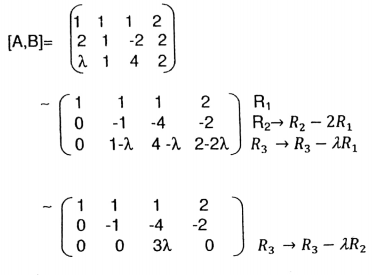Case [i]: ƛ = 0

⍴(A) = ⍴(A, B) = 2

The system is inconsistent and has infinitely many solutions.

Case [ii]: ƛ ≠ 0

⍴(A) = ⍴(A, B) = 3

The system is consistent and has a unique solution.

Question 57: Prove by vector method that cos (A – B) = cosA + cosB + sinA sin B.

Solution: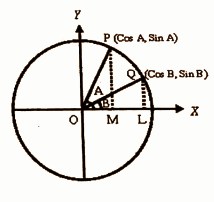Let |OP| = 1,|OQ | = 1

OP = cos A i + sin A j

OQ = cos Bi + sin Bj

OP . OQ = cos a . cos b + sin a . sin b

|OP||OQ|cos Ø = cos a . cos b + sin a . sin b

cos (a – b) = cos a . cos b + sin a . sin b

Question 58: Find the vector and cartesian equations of the plane through the points (1, 2, 3) and (2, 3, 1) and perpendicular to the plane 3x – 2y + 4z – 5 = 0.

Solution:

a = i + 2j + 3k

b = 2i + 3j + k

v = 3i – 2j + 4k

r = (1 – s) (i + 2j + 3k) + s (2i + 3j + k) + t (3i – 2j + 4k)

Cartesian equation is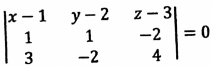(x – 1) (0) – (y – 2) (10) + (z – 3) (- 5) = 0

-10y + 20 – 5z + 15 = 0

-10y – 5z + 35 = 0

2y + z – 7 = 0

Question 59: ‘P’ represents the variable complex number z. Find the locus of P if Re [(z − 1) / (z + i)] = 1.

Solution:

Let z = x + iy

(z – 1) / (z + i)

= [(x + iy – 1) / (x + iy + i)]

= [(x – 1) + iy / (x + (y + 1) i]

= [(x – 1) iy / (x + i (y + 1))] [(x – i (y + 1)) / (x – i (y + 1))]

= [x (x – 1) + y (y + 1) + i (xy – (x + 1) (y + 1)] / [x2 + (y + 1)2]

Re [(z − 1) / (z + i)] = 1

(x (x – 1) + y (y + 1)) / [x2 + (y + 1)2] = 1

x2 – x + y2 + y = x2 + y2 + 2y + 1

x + y + 1 = 0

Question 60: A cable of a suspension bridge is in the form of a parabola whose span is 40 mts. The roadway is 5 meters below the lowest point of the cable. An extra support is provided across the cable 30 meters above the ground level. Find the length of the support if the height of the pillars is 55 mts.

Solution: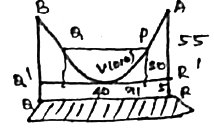Take the lowest point on the cable as the vertex that is the origin.

AB = 40m

R’A = 55m

RR’ = 5

Thus the point A is (20, 50).

The equation of the parabola is x2 = 4ay

202 = 4a (50)

a = 400 / 4 * 50

a = 2

The equation of the path x2 = 8y.

Let PQ be the vertical distance to the cable from the pole RQ. P is a point on the parabola.

x12 = 8 * 35

x1 = √4 * 2 * 35

= 10√2 m

The length of PQ = 2x1 = 20√2 m.

Question 61: Find the equation and eccentricity of the ellipse if:

i. the centre of the ellipse is the same as the center of the hyperbola 4x2 – 9y2 + 8x – 36y + 68 = 0

ii. length of the semimajor axis is 3

iii. length of the minor axis is 2

iv. the equation of the major axis is x = -1.

Solution:

The equation of hyperbola is 4x2 – 9y2 + 8x – 36y – 68 = 0

Center of hyperbola = (-1, -2)

Center of ellipse = (-1, -2)

Length of semi-major axis = 3

Length of the minor axis = 1 = b

Length of semi-minor axis = 2

Equation of ellipse = (x + 1)2 / 9 – (y + 2)2 / 4 = 1

Eccentricity = e = √(1 – (b2 / a2)

= √1 – (1 / 9)

= √8 / 8

= 2√2 / 3

The equation of the ellipse is (x + 1)2 / 1 + (y + 2)2 / 9 = 1.

Question 62: Find the equation of the rectangular hyperbola which passes through the points (6, 0) and (-3, 0) and has an asymptote x + 2y – 5 = 0.

Solution:

One of the asymptotes is x + 2y – 5 = 0.

The two asymptotes are perpendicular and intersecting another equation of the asymptote 2x – y + k = 0.

The combined equation of the asymptotes is (x + 2y – 5) (2x – y + k) = 0.

The equation of the asymptotes differ by only a constant (x + 2y – 5) (2x – y + k) + k = 0 passing through the points (6, 0) and (-3, 0).

(-8) (6 + k) + c = 0

48 – 8k + c = 0 —- (1)

(12 + k) + c = 0 —- (2)

Taking (1) and (2),

-9k = -36

k = 4

Substitute k = 4 in (1),

48 – 64 + c = 0

c = 16

The equation of the rectangular hyperbola (x + 2y – 5) (2x – y + 4) + 16 = 0.

Question 63: Let P be a point on the curve y = x3 and suppose the line tangent line P intersects the curve again at Q. Prove that the slope at Q is four times the slope at P.

Solution: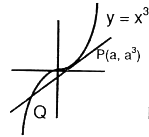y = x3

y’ = 3x2

When x = a, m1 = 3a2

P is point on the tangent at I.

Thee equation of the tangent (y – y1) = m (x – x1)

y – a3 = 3a2 (x – a)

y – a3 = 3xa2 – 3a3

x3 – 3a2x + 2a3 = 0

When y = x3, x = a, x = -2a

The line touches the curve again at point Q.

The slope of Q is m2 = 3x2

= 3(-2a)2

= 3 * 4a2

= 12a2

The slope at Q is 4 times the slope at P.

Question 64: Trace the curve y = x3.

Solution: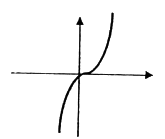y = x3

The curve defined (-∞, ∞).

Domain = (-∞, ∞)

Extension x ⇒ -∞ < x < ∞

y ⇒ -∞ < y < ∞

Intercept

x ⇒ y = 0

y ⇒ x = 0

The curve passing through the origin: symmetry test: x is -x, y is – y.

The curve doesn’t change the symmetry about both and the origin.

Asymptotes

The curve doesn’t admit any asymptotes.

Monotonicity: f (x) = x3

f’ (x) = 3x2

f’(x) > 0 is real

The point of inflection is (0, 0).

Question 65: Find the common area enclosed by the parabolas 4y2 = 9x; 3x2 = 16y.

Solution:

4y2 = 9x —- (1)

3x2 = 16y —- (2)

On solving the above equations,

x2 = 8√x

x4 = 64x

x (x3 – 64) = 0

x = 0, 4

When x = 0, y = 0 and x = 4, y = 3.

Let f (x) = 3√x / 2

g (x) = 3x2 / 16

The curve intersection point is (10, 0) and (4, 3) about the x-axis.

Required area = ∫04 [f (x) – g (x)] dx

= ∫04 [(3√x / 2) – (3x2 / 16)] dx

= [x3/2 – x3 / 16]04

= 4√4 – (64 / 16)

= 8 – 4

= 4 square units

Question 66: Find the length of the curve (x / a) + (y / a) = 1.

Solution:

The parametric equations are x = acos3t and y = asin3 t.

dy / dt = 3asin2t cost

dx / dt = -3a cos2t sint

(dx / dt)2 + (dy / dt)2 = 9a2 cos2t sin2t

√(dx / dt)2 + (dy / dt)2 = 3a sint cost = (3a / 2) sin2t

t varies from 0 to ?? / 2 in the first quadrant.

Length = 4 * [length of 0 to 𝛑 / 2 arc]

= 4 ∫0𝛑/2 √(dx / dt)2 + (dy / dt)2 dt

= 4 ∫0𝛑/2 (3a / 2) sin2t dt

= 6a ∫0𝛑/2 sin2t dt

= 6a [-cos 2t / 2]0𝛑/2

= 6a

Question 67: Solve: (x3 + 3x2)dx + (y3 + 3x2y)dy = 0.

Solution:

(x3 + 3x2)dx + (y3 + 3x2y)dy = 0

x3 dx + y3 dy + 3xy (x dy + y dx) = 0

∫x3 dx + ∫y3 dy + 3 ∫d (xy)2 / 2 = 0

(x4 / 4) + (y4 / 4) + (3 / 2) (xy)2 = c

x4 + y4 + 6x2y2 = c

Question 68: The rate at which the population of a city increases at any time is proportional to the population at that time. If there were 1,30,000 people in the city in 1960 and 1,60,000 in 1990, what approximate population may be anticipated in 2020? [loge [16 / 13]] = 0.2070, e0.42 = 1.52]

Solution:

Let x be the population of the city at time t.

If t = 0, at 1960, x0 = 130000

dx / dt = kx

∫dx / x = ∫k dt

log x – log c = kt

x / c = ekt

x = cekt

t = 0, x = 130000

130000 = c(1)

X = (130000) ekt

At t = 30, mean in 1990

x = 160000

16 = 13 ekt

ekt = 16 / 13

kt = ln (16 / 13)

k = 1 / 30 ln (16 / 13) = 0.0069

In 2020 t = 60

x = 130000 e60*0.0069

x = 130000 e0.42

x = 197600

Question 69: If the number of incoming buses per minute at a bus terminus in a random variable having a Poisson distribution with 𝜆 = 0.9, find the probability that there will be:

i. Exactly 9 incoming buses during a period of 5 minutes.

ii. Fewer than 10 incoming buses during a period of 8 minutes.

iii. At Least 14 incoming buses during a period of 11 minutes.

Solution:

[i] Let 𝜆 be the number of incoming buses per minute = 0.9

𝜆 for the number of incoming buses per 5 minutes = 4.5

P (exactly 9 incoming buses during a period of 5 minutes) = [e-𝜆 𝜆9] / 9!

P (X = 9) = e-4.5 (4.5)9 / 9!

[ii] P (fewer than 10 incoming buses during a period of 8 minutes) = P (X < 10)

𝜆 = 0.9 * 8 = 7.2

P (X < 10) = ∑x=09 e-7.2 (7.2)x / x!

[iii] P (at least 14 incoming buses during a period of 11 minutes) = P (X ≥ 14)

= 1 – P (X < 14)

= 1 – ∑x=013 e-9.9 (9.9)x / x!

Question 70: [a] Show that the volume of the largest right circular cone that can be inscribed in a sphere of radius ‘a’ is 8 / 27 (volume of the sphere).

OR

[b] With usual notations, show that (Zn, +n) forms a group.

Solution:

[a]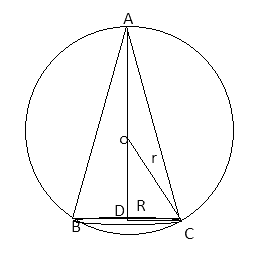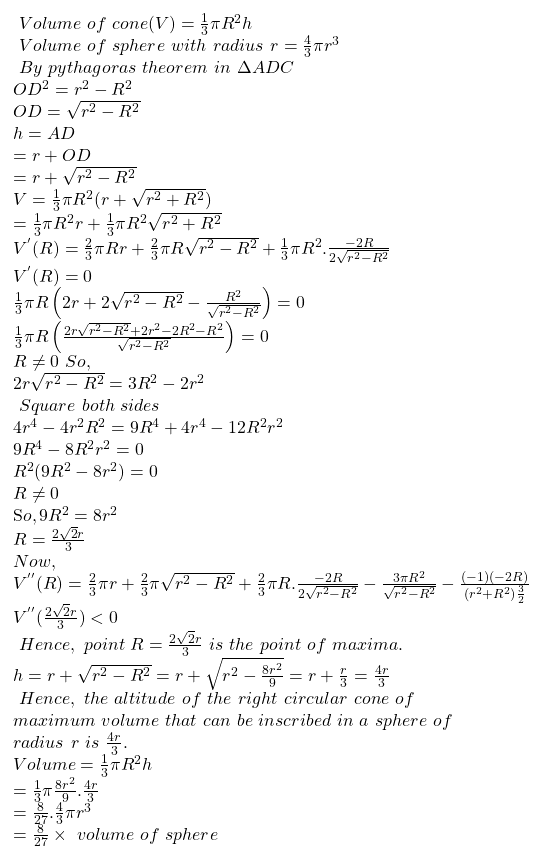OR

[b] Zn = [{0}, {1}, ….. {n – 1}]be the set of all congruent classes of modulo n.

[l], [m] ∈ Zn

0 ≤ 1, m < n

[i] closure

[l] + [m] = {[l + m] if l + m < n

[r] l + m ≥ n

where l + m = q . n + r, 0 ≤ r ≤ n

[l + m], [r] ∈ Zn

Closure axiom is true.

[ii] Addition modulo n is always associative in the set of congruence classes modulo n.

[iii] The identity element  ∈ Zn

[iv] The inverse of [l] ∈ Zn

[n – l] ∈ Zn

[l] + [n – l] =  [n – l] + n [l] = 

The inverse axiom is true.

So, (Zn, +n) forms a group.